# Symmetric Key Algorithm

Hacker Dōjo Workshop：
Type: crtptography
Grant：200 USDT
Bounty：https://dorahacks.io/daobounty/193
Dojo hacker：vyshnav
WeChat: @HackerDojo0
E-mail: hackerdojo0@gmail.com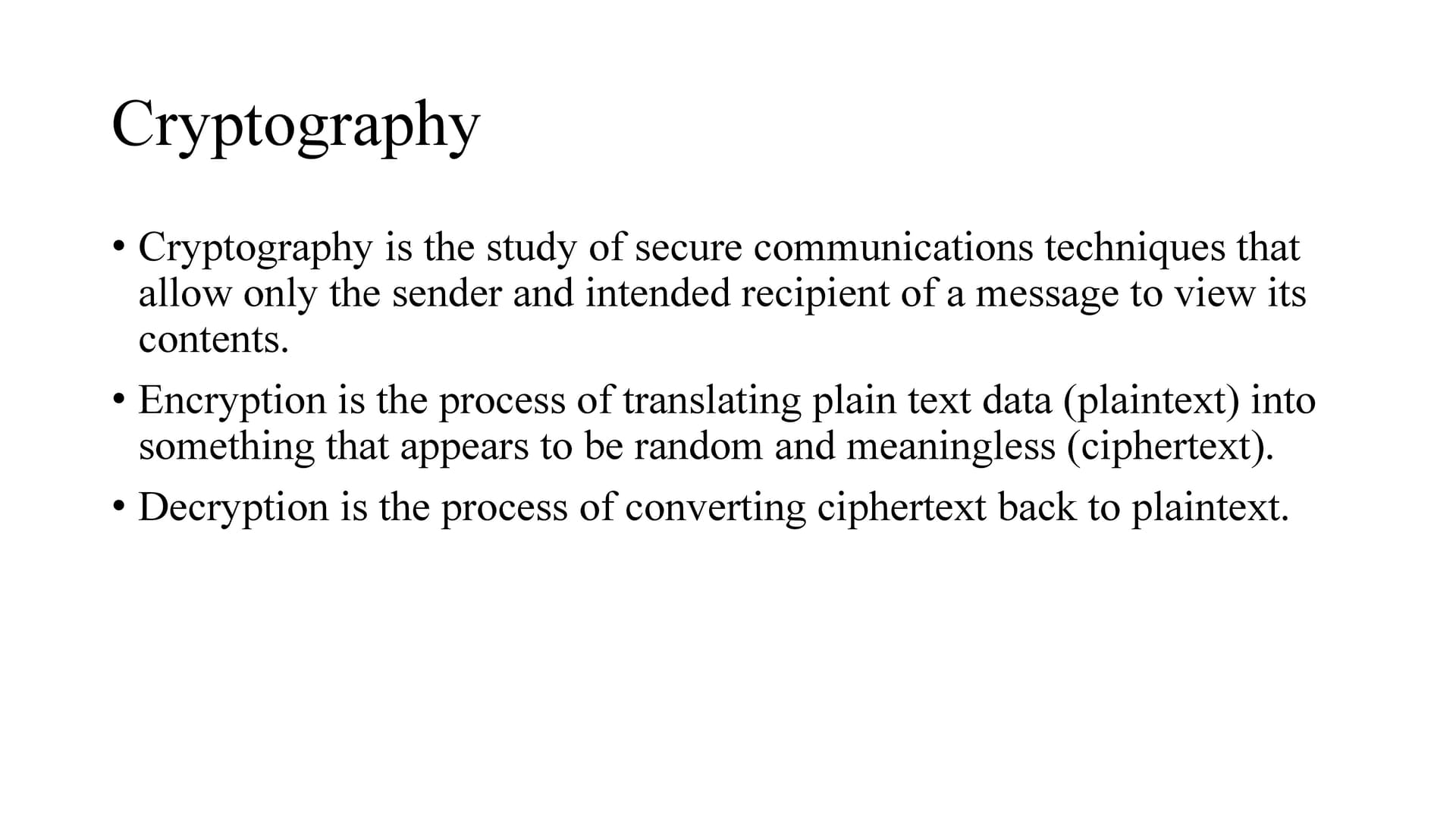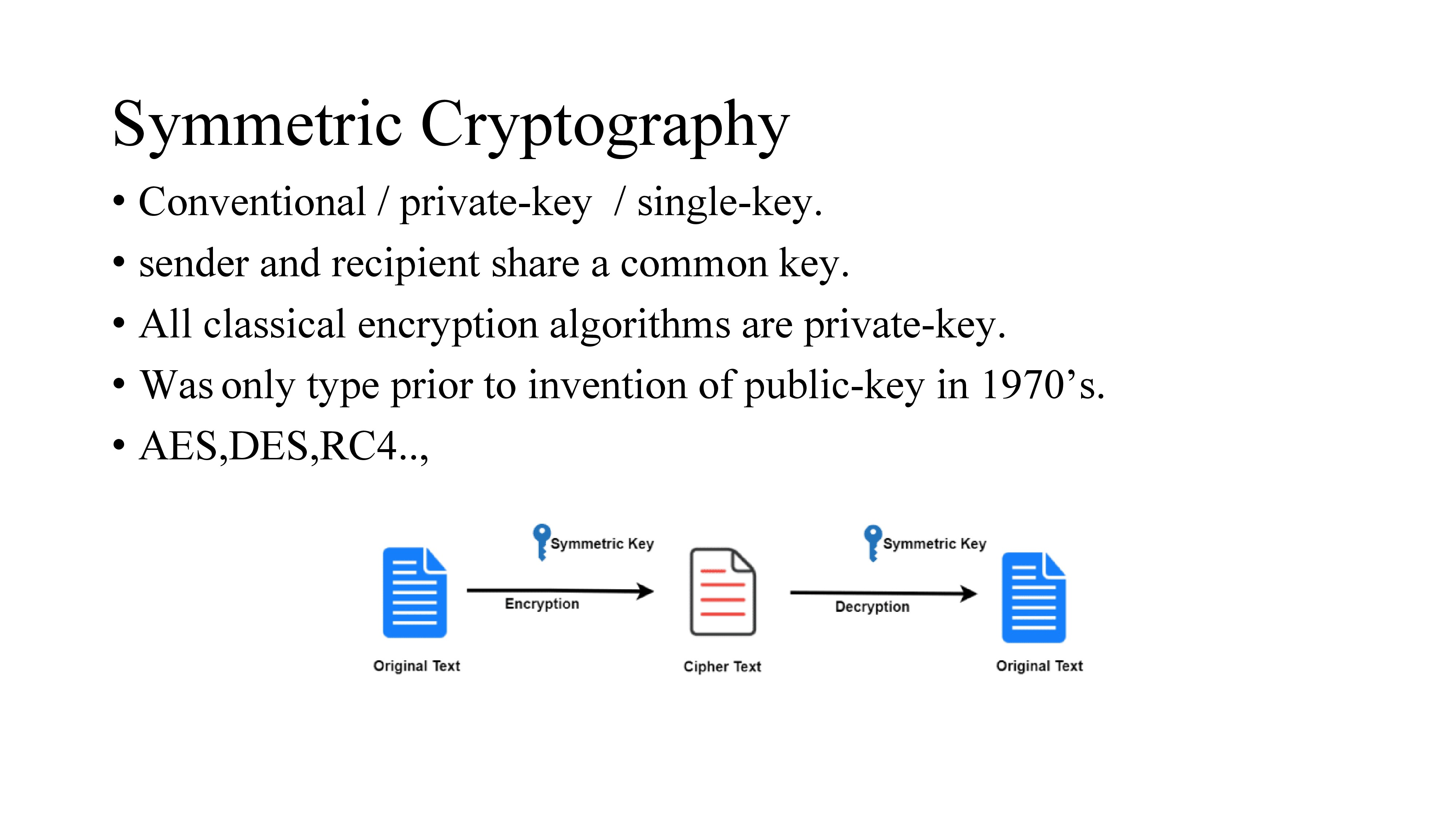What is Symmetric key algorithm?
A cryptographic algorithm that uses the same secret key for encrypting as well as decrypting.
It is also called Conventional / private-key / single-key.
Here, sender and recipient share a common key i.e. Sender encrypts data with a key and send the cipher text to receiver. Receiver decrypts the data with the same key to get plain text.
Keys are generated using random number generators or pseudo random number generators.
And the key is distributed using various key distribution methods like Public announcement, Publicly available directory, Public-key authority.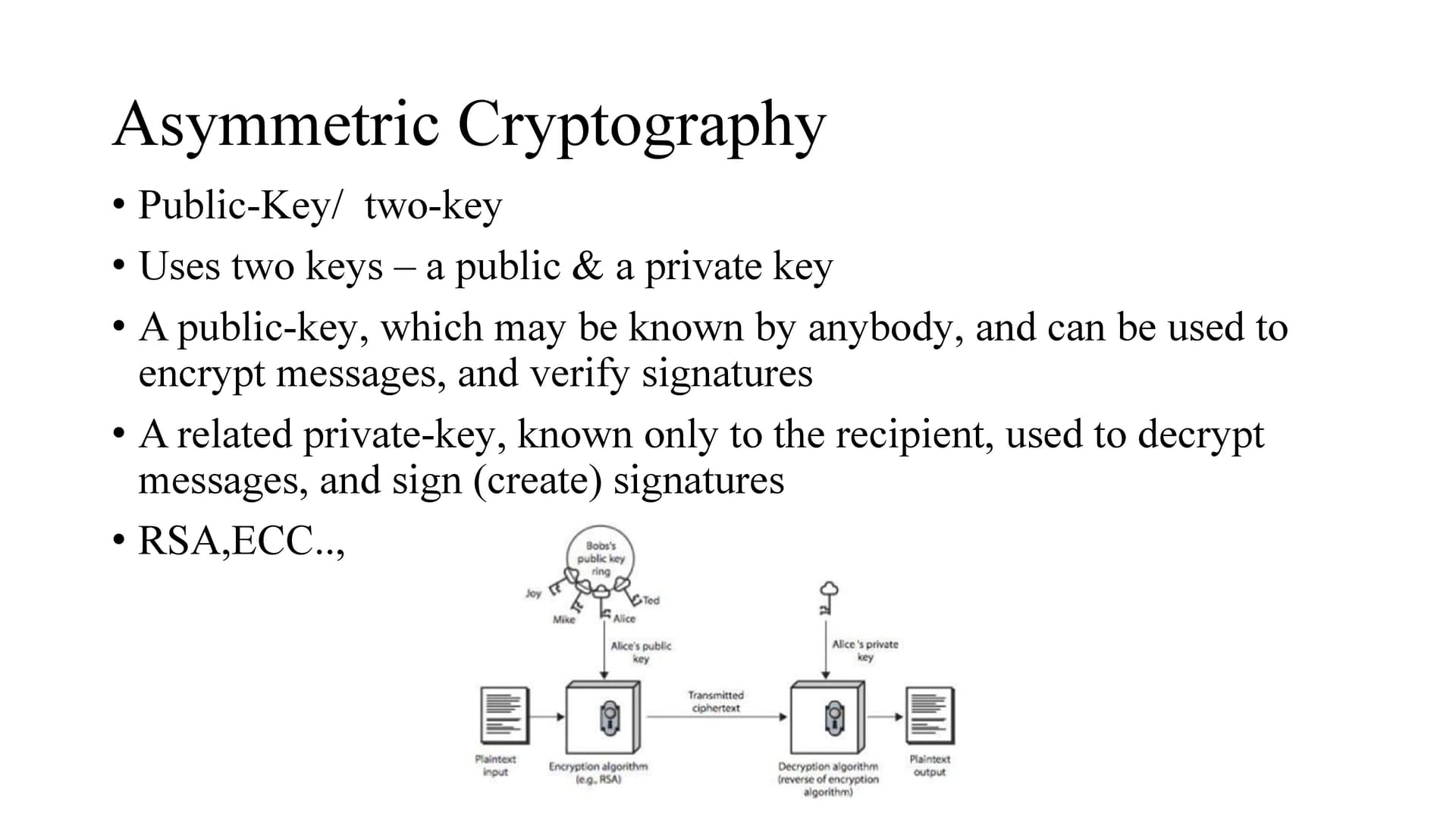Asymmetric cryptography is a process that uses a pair of related keys – one public key and one private key – to encrypt and decrypt a message.
Private key should be kept confidential and public key is generated using private key.
A public-key, can be used to encrypt messages and private key is used to decrypt the message.
In the picture we can see, Bob wants to encrypt the data and send it to alice. So bob takes alice public key and encrypt the data with it. And send it to alice. As the data is encrypted with alice public key, only alice can decrypt it.

The same public key and private key concept is used for digital signatures.
But it works the other way around.
Private key is used to sign the document and public key is used to verify the signature of the document.
Bob signs a document with his private key and anyone can authenticate that using bobs public key. Digital signature provides integrity.
The main advantage of symmetric encryption over asymmetric encryption is that it is fast and efficient for large amounts of data; the disadvantage is the
need to keep the key secret.
There are misconceptions about asymmetric key encryption like asymmetric key encryption is more secure.
But the truth is, the security is based on key size if the key size is more it is difficult to brute force.
Another misconception is
public- key encryption is a general-purpose technique that has made symmetric encryption obsolete.
But, because of the computational overhead of current public-key encryption schemes, there seems no foreseeable likelihood that symmetric encryption will be abandoned.
As one of the inventors of public key encryption has told “the restriction of public key cryptography to key management and signature applications is universally accepted”.
So asymmetric encryption can be used to transmit the key of symmetric encryption .
So shared key for symmetric key encryption can be shared using Key distribution mechanisms or asymmetric key encryption.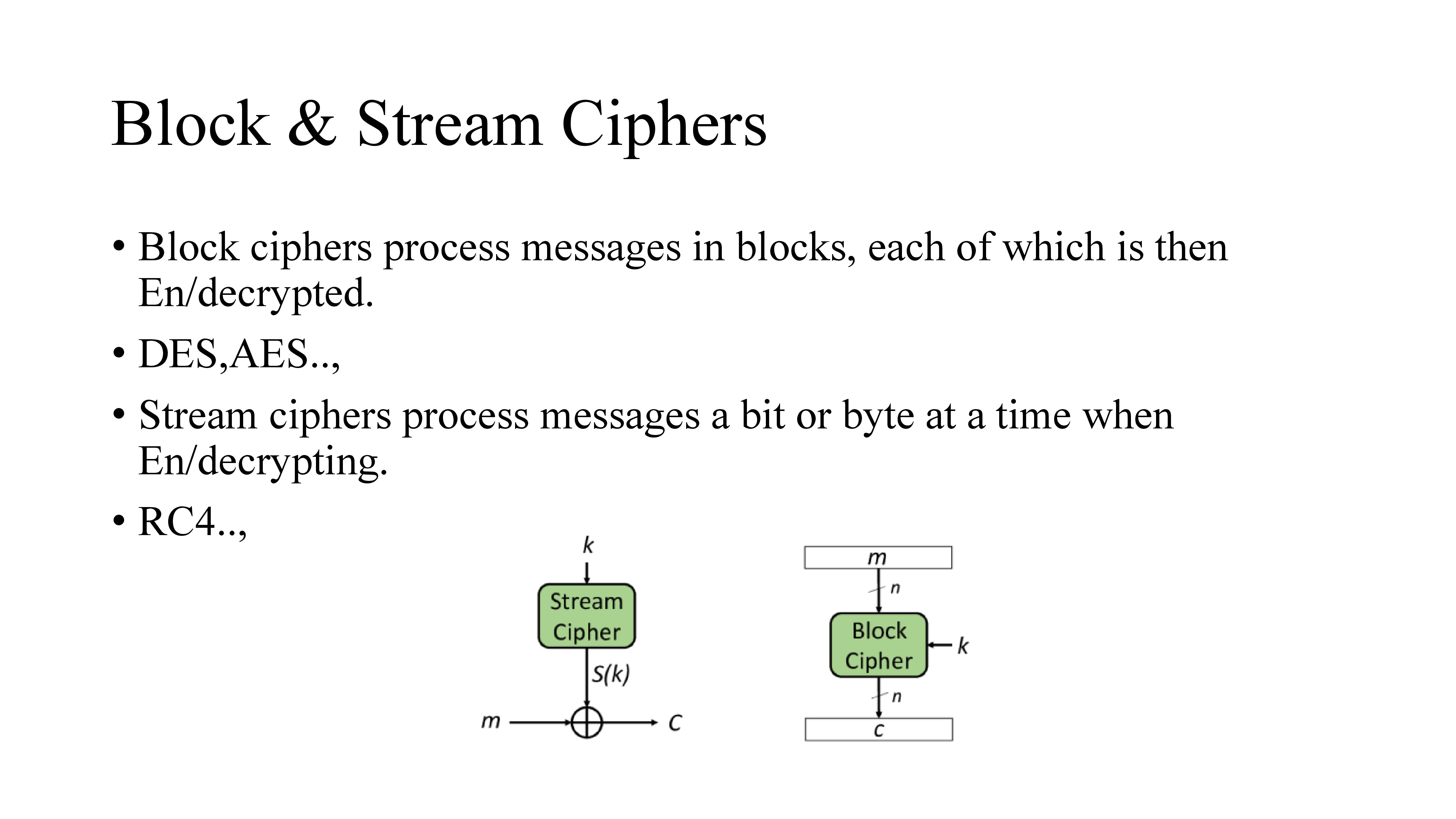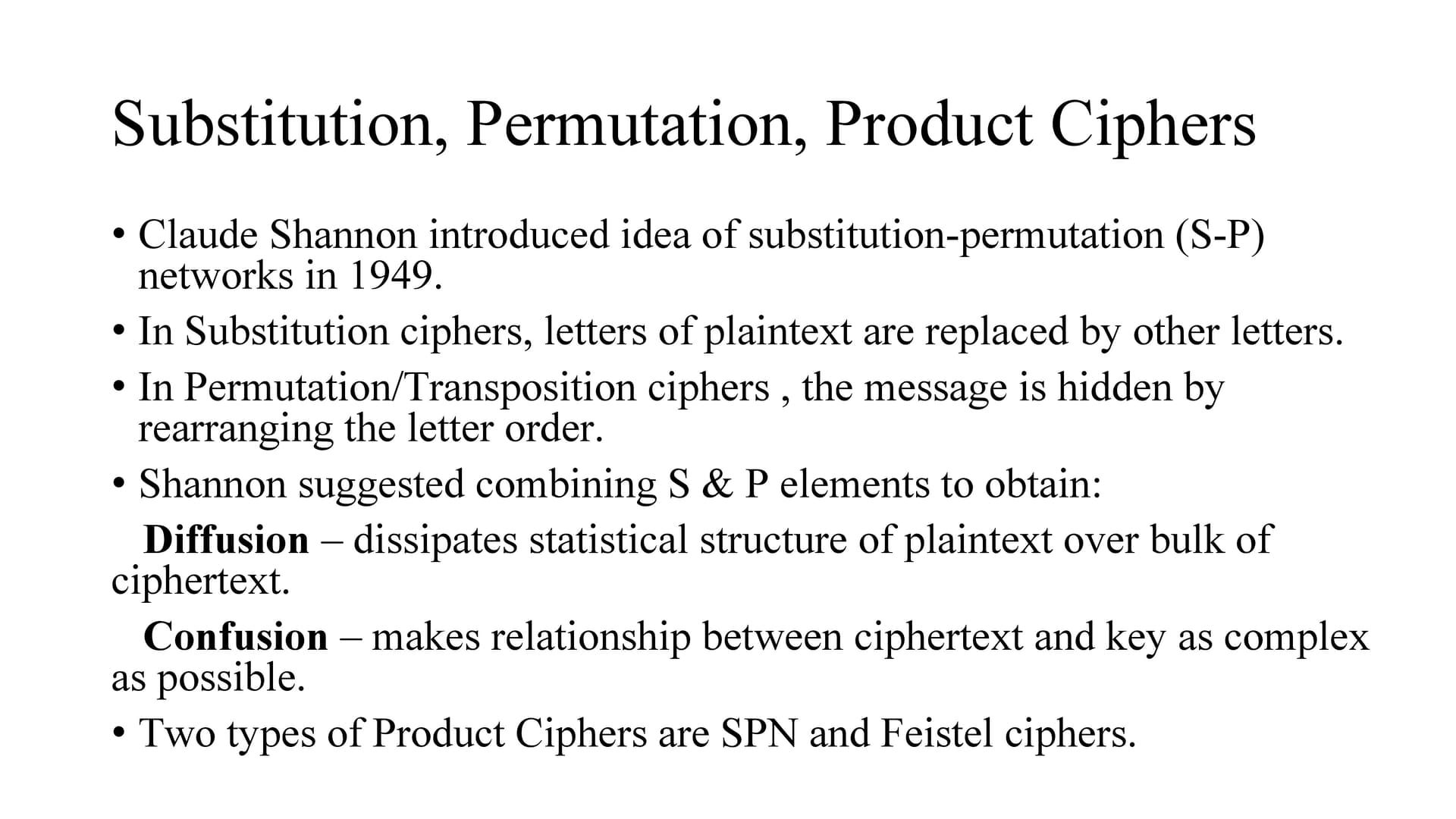There are two common techniques used to construct ciphers i.e. cryptographic algorithms: substitution and permutation.
In Substitution ciphers, letters of plaintext are replaced by other letters.
In Permutation/Transposition ciphers , the message is hidden by rearranging the letter order.
But Claude Shannon in the year 1949 introduced idea of substitution-permutation (S-P) networks .
He suggested combining S & P elements to obtain:
Diffusion – dissipates statistical structure of plaintext over bulk of ciphertext.
Confusion – makes relationship between ciphertext and key as complex as possible.
Claude Shannon’s 1949 paper has the key ideas that led to the development of modern block ciphers.
Feistel proposed the use of a cipher that alternates substitutions and permutations, as a practical application of a proposal by Claude Shannon leads to product cipher.
Its like utilizing the concept of a product cipher, which is the execution of two or more simple ciphers in sequence in such a way that the final result is cryptographically stronger.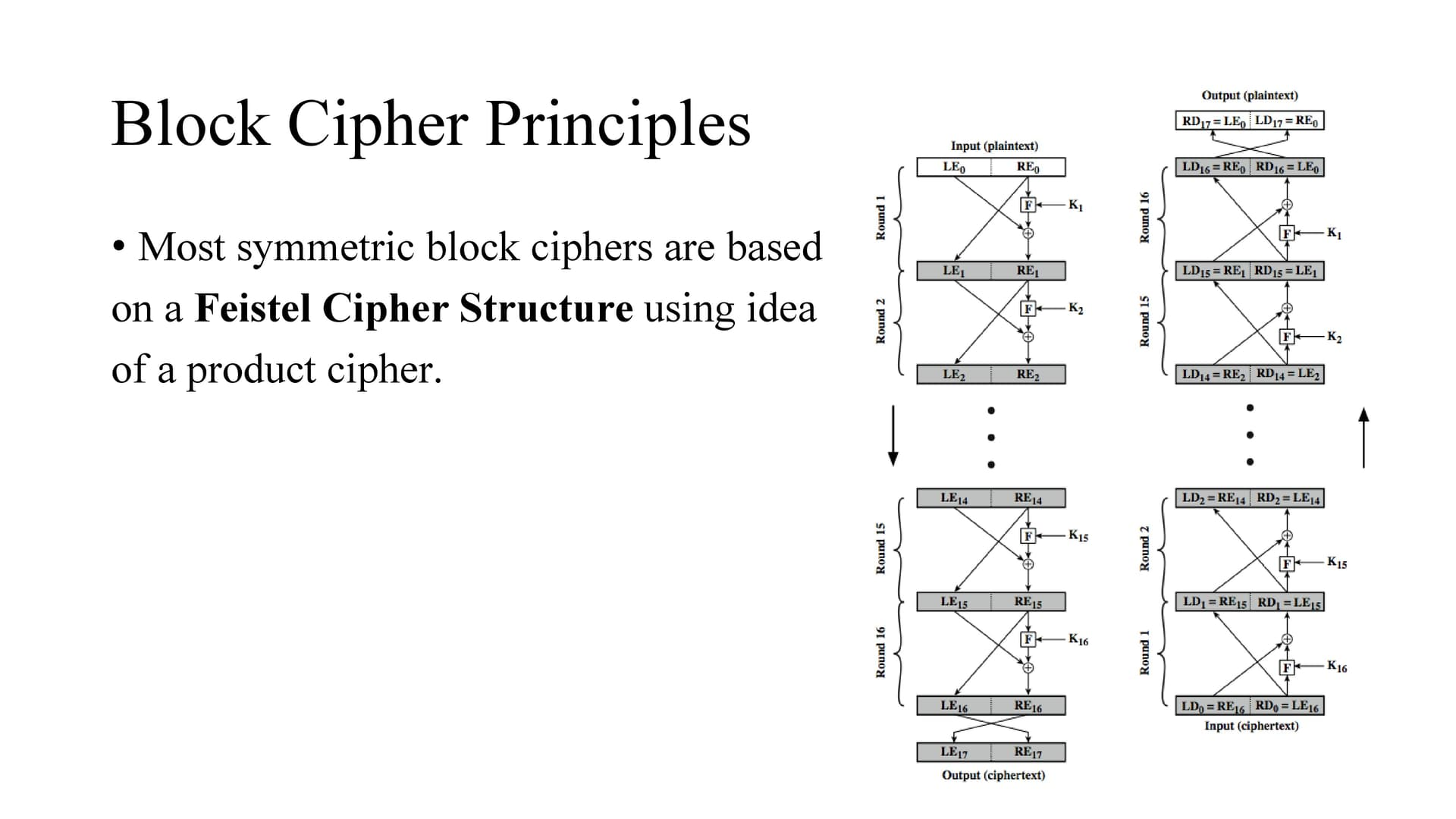In the feistel cipher structure, the data is split into 2 halves, processed through a number of rounds which perform substitution and permutations.
The LHS side of this figure shows the flow during encryption, the RHS in decryption.
The process of decryption with a Feistel cipher is the same as the encryption process. Use the ciphertext as input to the algorithm, but use the subkeys Ki in reverse order.
That is, use Kn in the first round, Kn–1 in the second round, and so on until K1 is used in the last round. Here we need not implement two different algorithms,
one for encryption and one for decryption. The intermediate value of the decryption process is equal to the corresponding value of the encryption process with the two halves of the value swapped.In the late 1960s, IBM set up a research project Feistel. The project concluded in 1971 with the development of the LUCIFER algorithm.
LUCIFER is a Feistel block cipher that encrypts blocks of 64 bits, using a key size of 128 bits.
Later, to develop a marketable commercial encryption product that ideally could be implemented on a single chip they produced a a refined version of LUCIFER that had a reduced key size of 56 bits, to fit on a single chip.
In 1973, the National Bureau of Standards (NBS) issued a request for proposals for a national cipher standard. IBM submitted the modified LUCIFER and was was eventually accepted as the DES.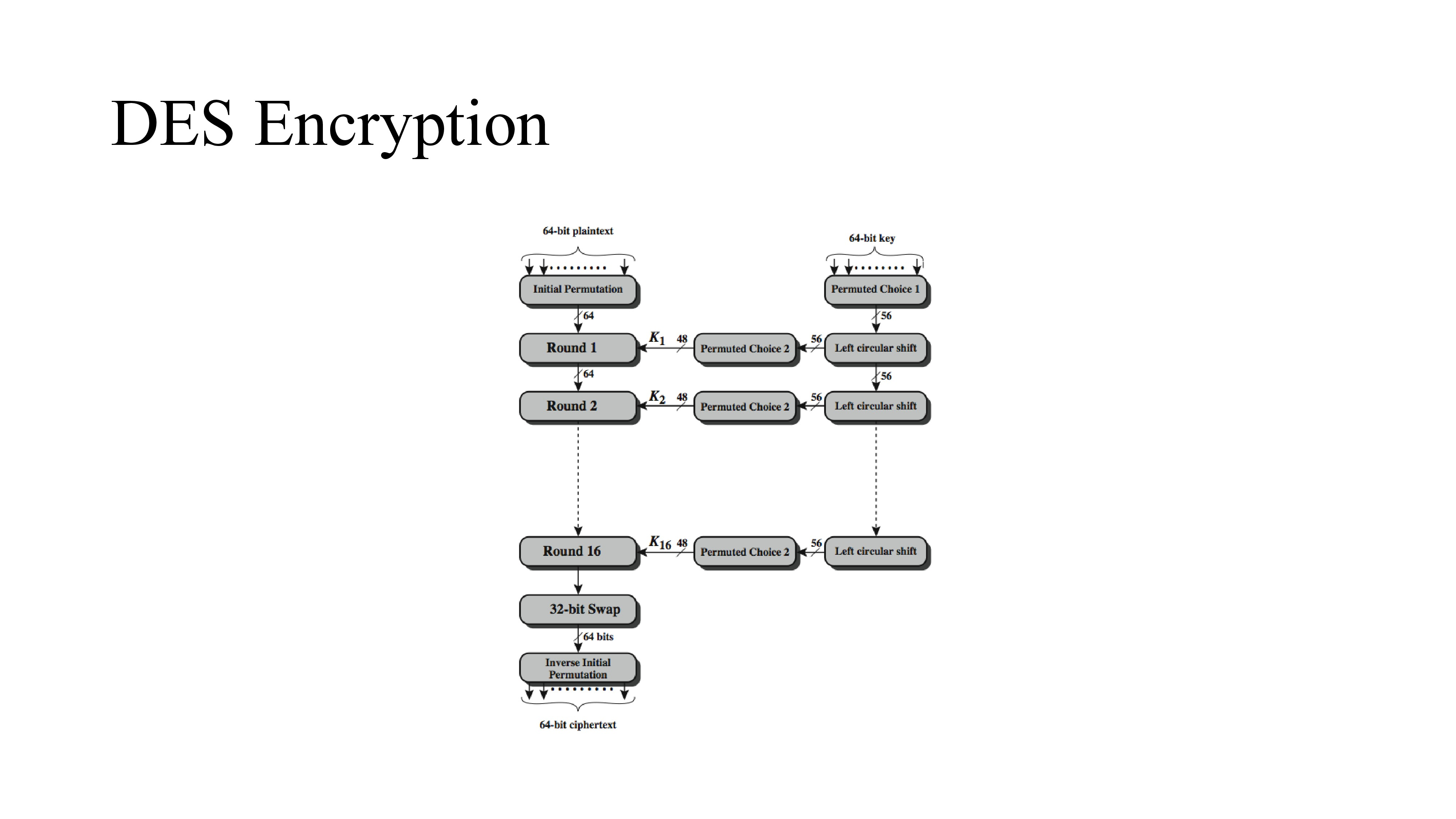DES Encryption takes input as 64 bit data and 64 bit key.
The left side shows the basic process for enciphering a 64-bit data block
It consists of:

• an initial permutation (IP) which shuffles the 64-bit input block
• 16 rounds of a complex key dependent round function that involves substitutions & permutations
• a final permutation, being the inverse of IP
The right side shows the handling of the 56-bit key and consists of:
• an initial permutation of the key (PC1) which selects 56-bits out of the 64-bits input, in two 28-bit halves
• 16 stages to generate the 48-bit subkeys using a left circular shift and a permutation of the two 28-bit halves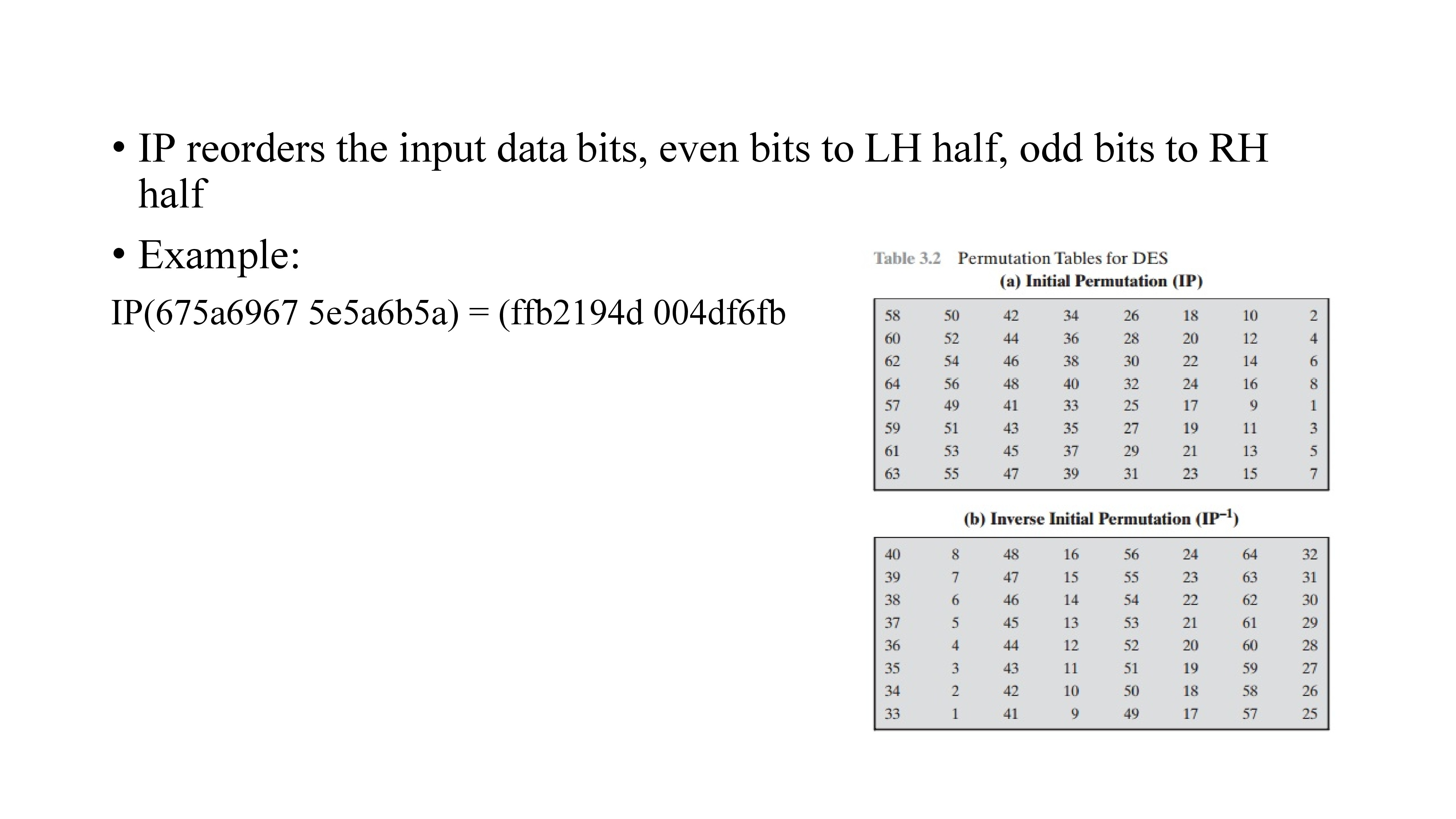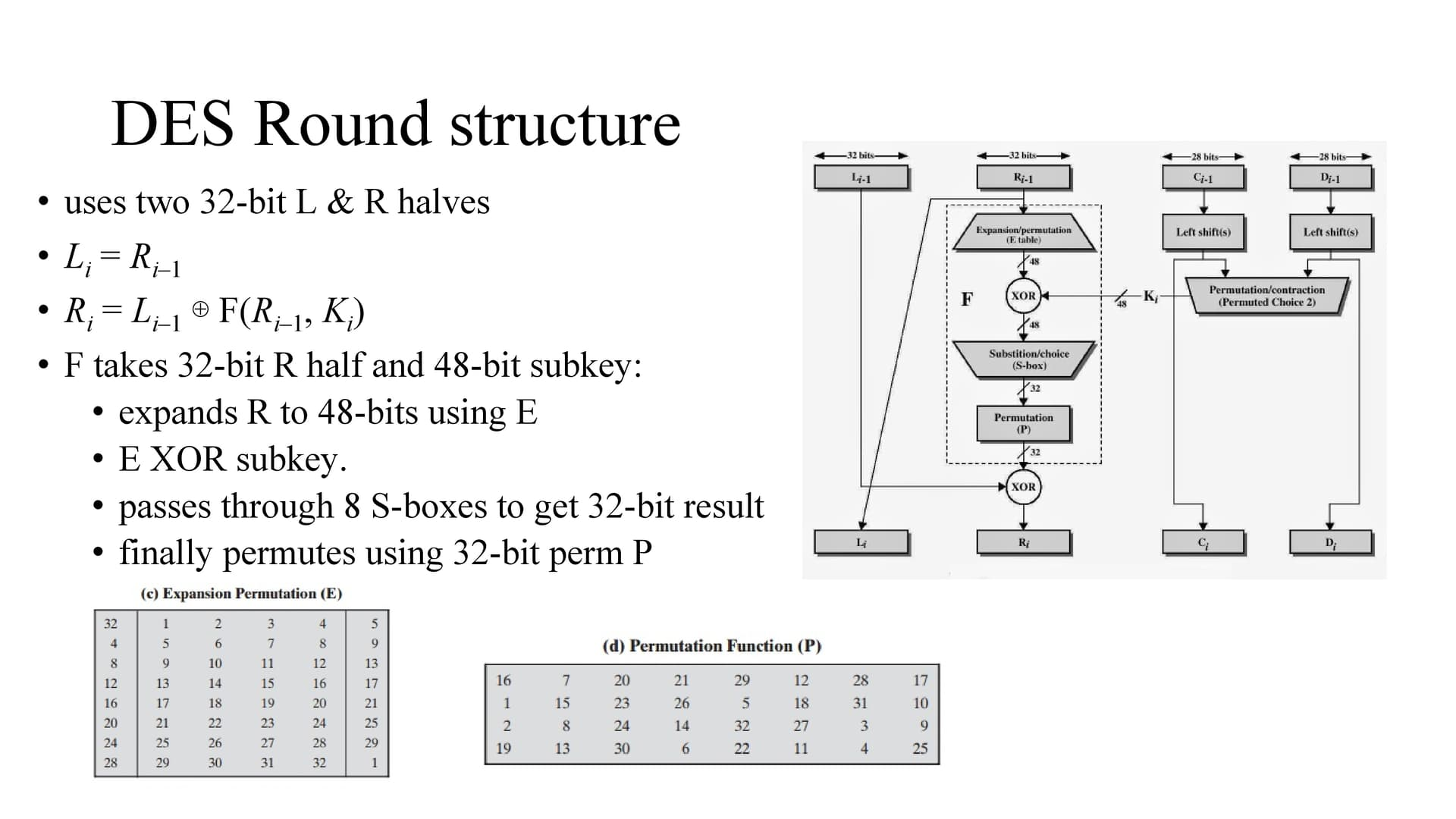AS we know, the input data is 64 bits and after initial permutation the data is divided into two halves left and right.
consider li-1 and ri-1 is current step, li and ri as next step
So li is same as ri-1 and the ri is Li–1 ⊕ F(Ri–1, Ki)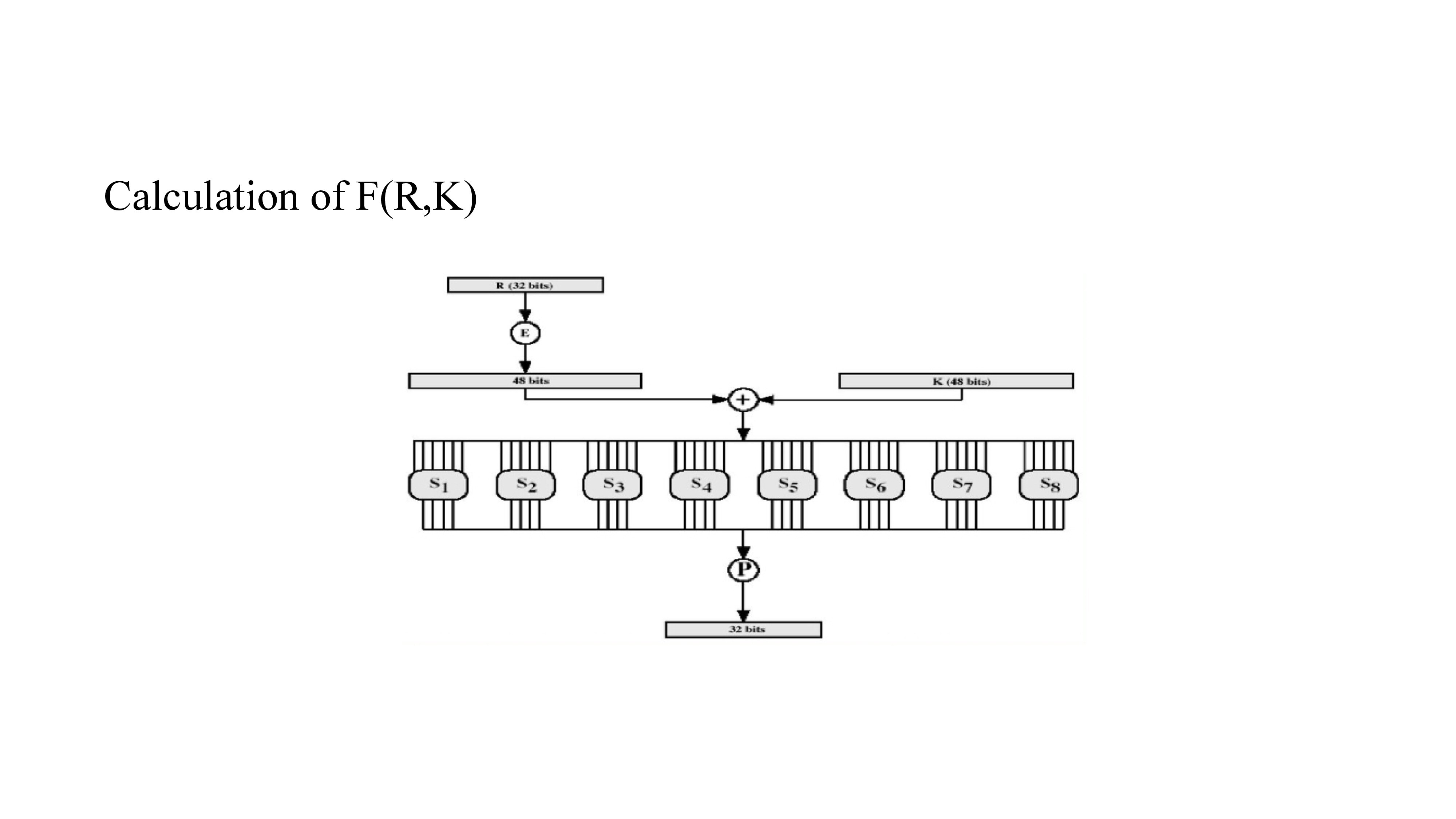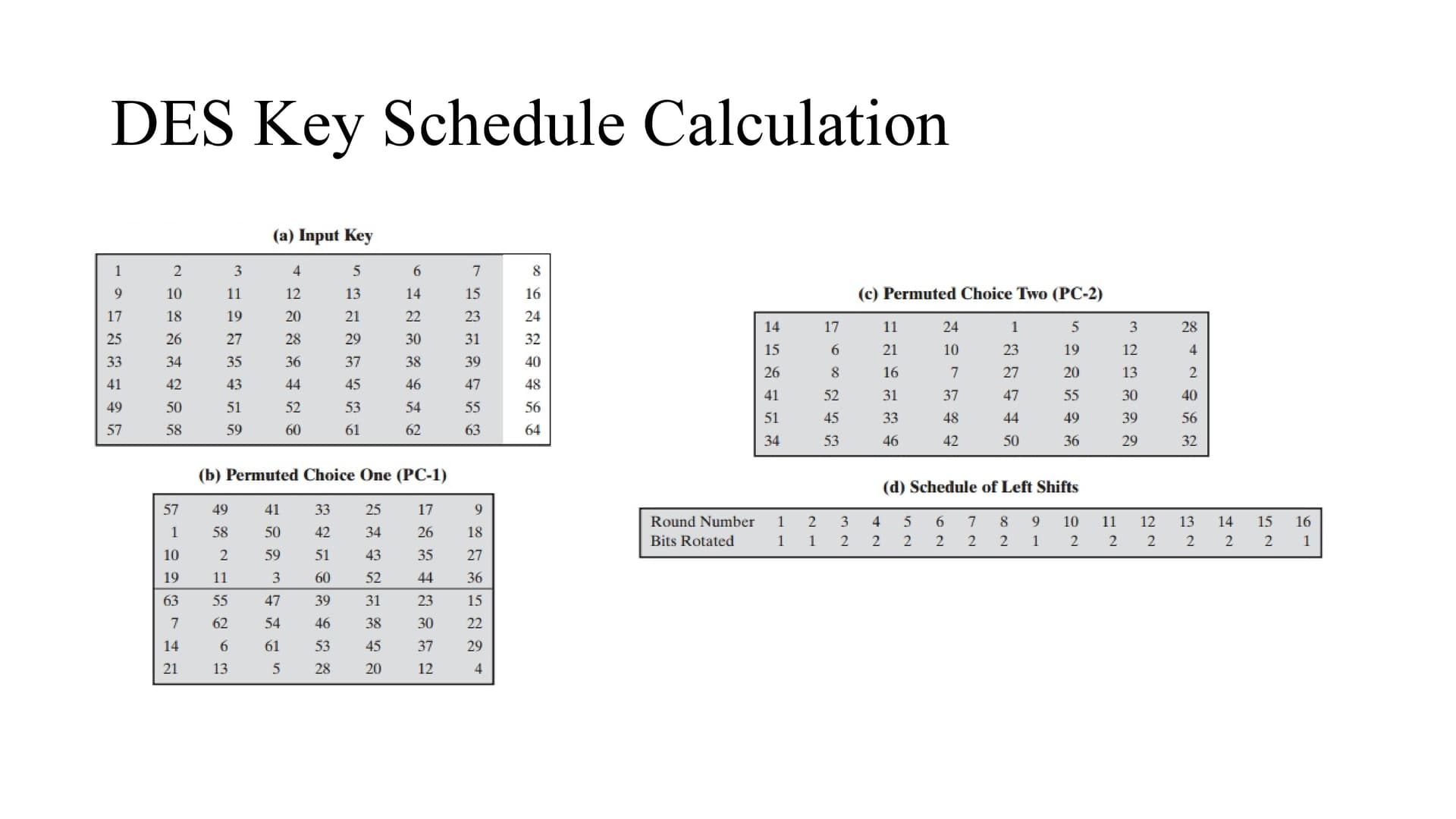At first the input key is 64 bits and it got reduced to 56 bits i.e. as we can see in the figure the bits 8,16,24,…64 are not considered.
Permutated choice 1 (PC1) simply rearranges the bits position according to figure b.
As we can see in figure d the left shift is based on the number of the round i.e. if it is 1 round , the bits are left circular shifted by 1, if it is 3rd round the left circular shift is by 2 bits and so on.
Permutated choice 2 (PC2) simply rearranges the bits position according to figure c.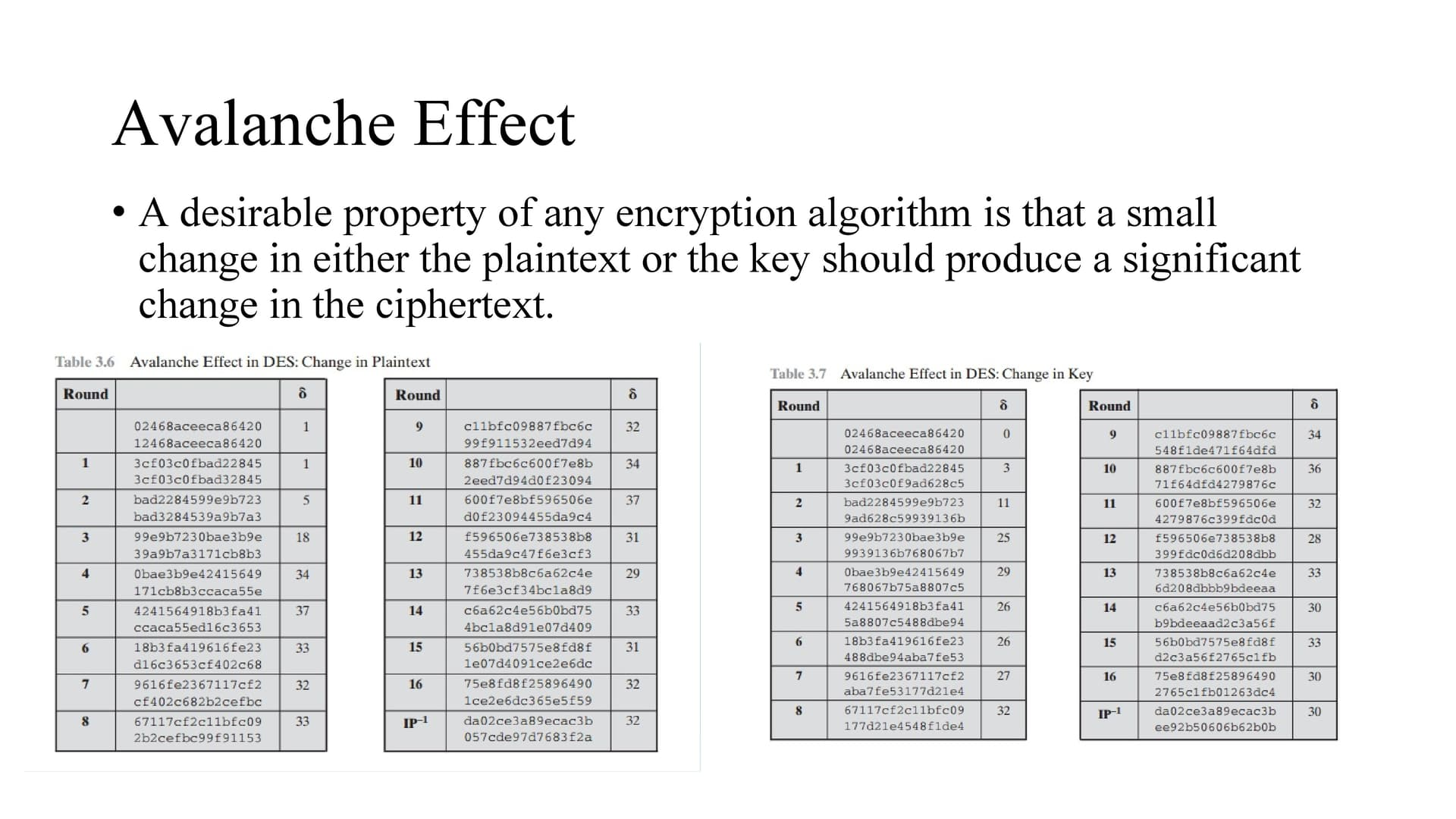So changing a single bit of plaintext changes 32 bits in the output after passing through the algorithm.
Similarly changing a single bit of key changes 30 bits in the output after passing through the algorithm.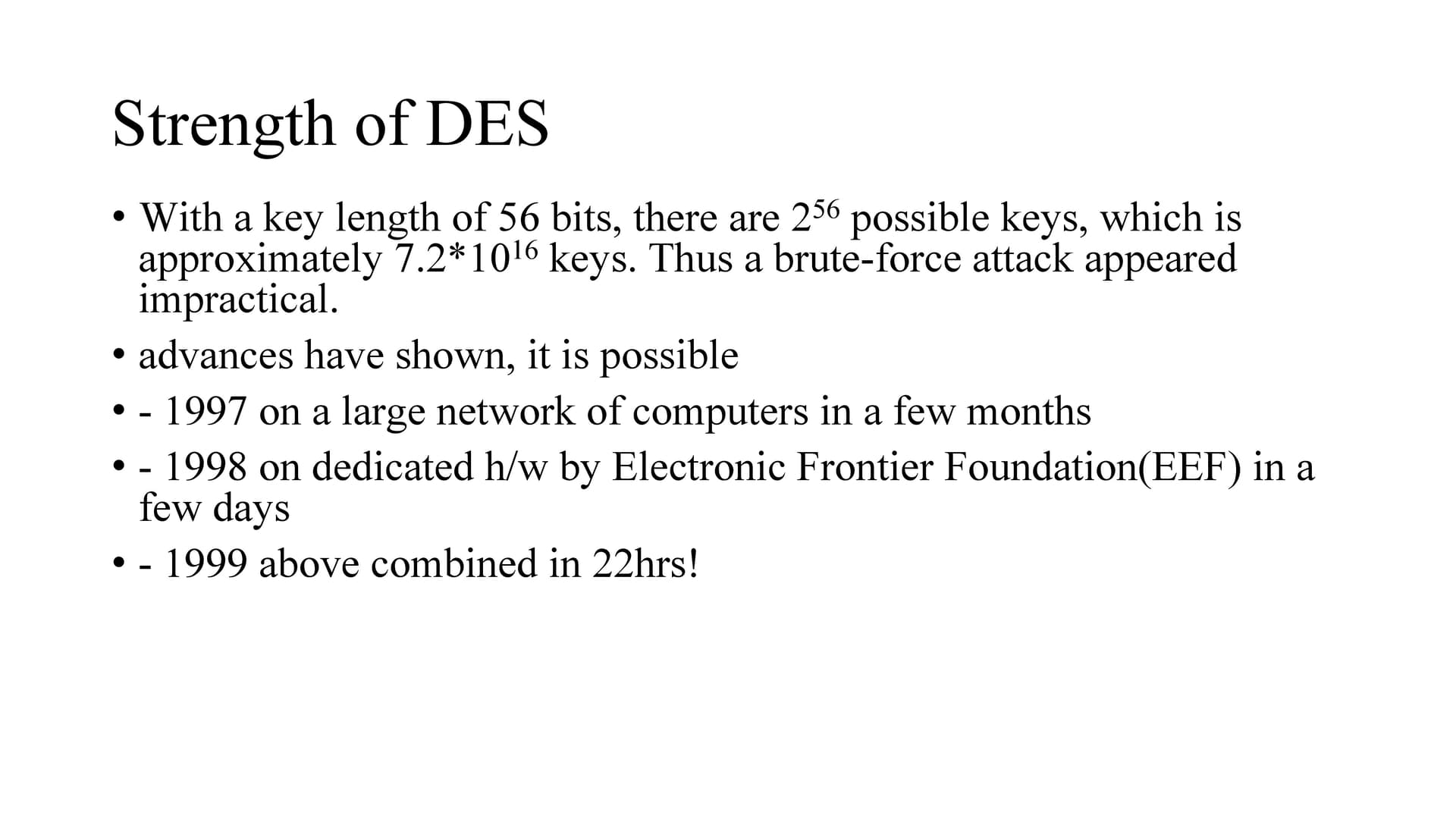When DES was made a standard the brute force attack appeared impractical.
But in future it was cracked.
In 1997 on a large network of computers cracked DES in a few months.
in July 1998, the Electronic Frontier Foundation (EFF) announced that it had broken a DES encryption using a special-purpose “DES cracker” machine that was built for less than \$250,000. The attack took less than three days.
In 1999 combining DES Cracker and a network of computers des cracked in 22hrs!
SO alternatives has to be considered.
3DES is one.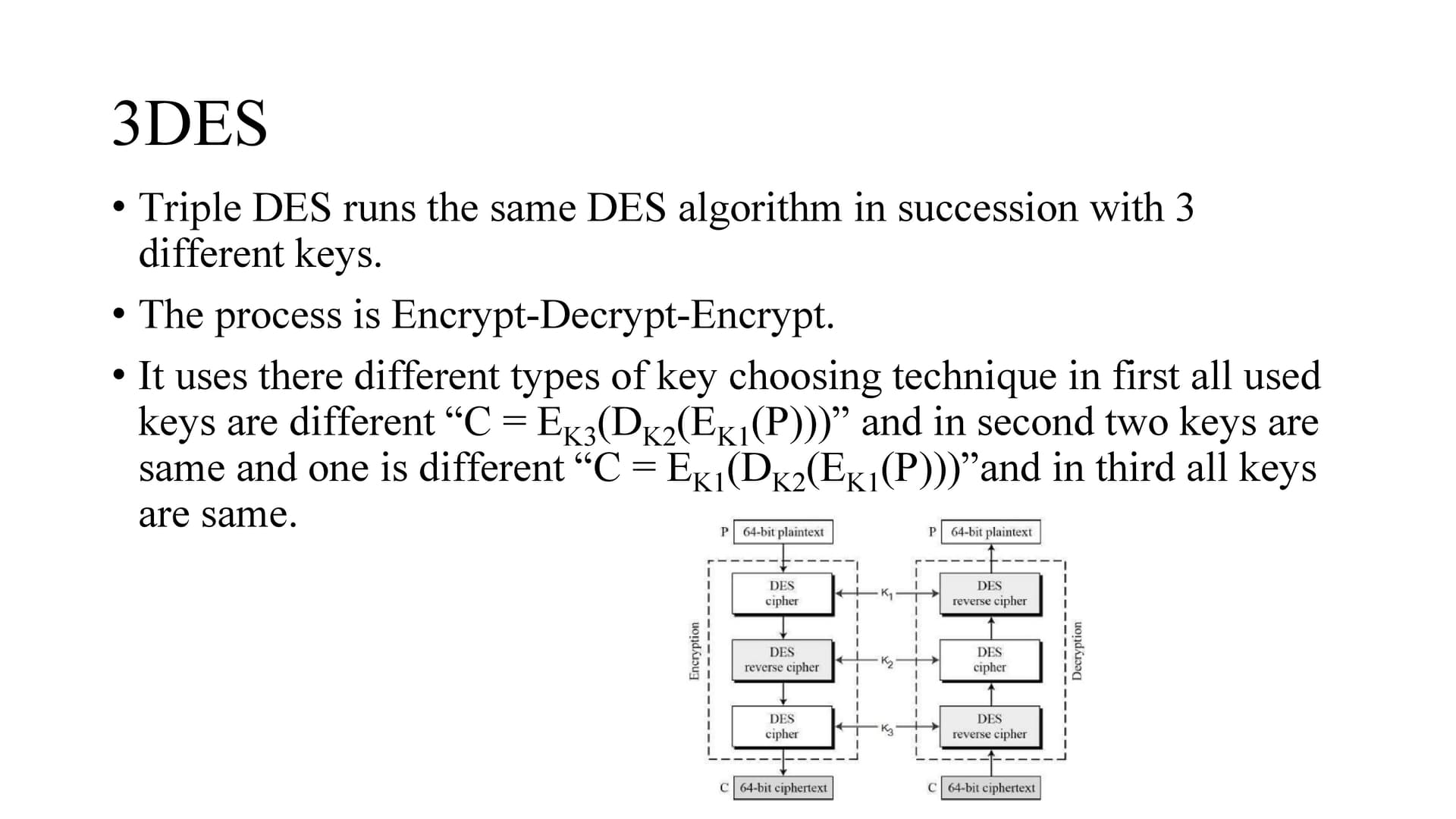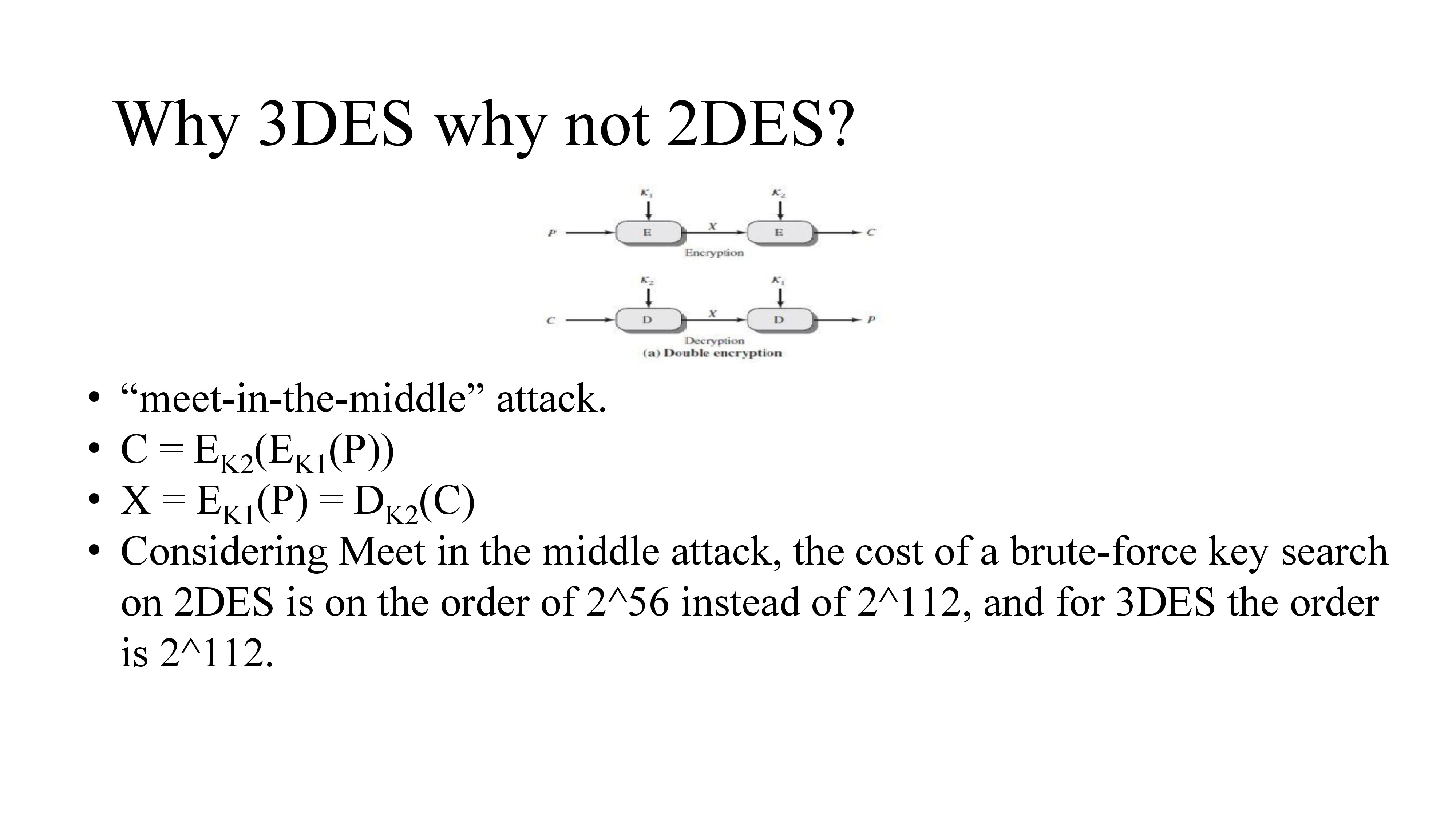Meet in the middle attack is a “known plain text” attack where attacker knows one or more plaintext ciphertext pairs formed with the secret key.
So the attacker brute force all possible keys to find x in both encryption and decryption and check if x value is same for both. If the x value is same then the attacker got both k1 and k2.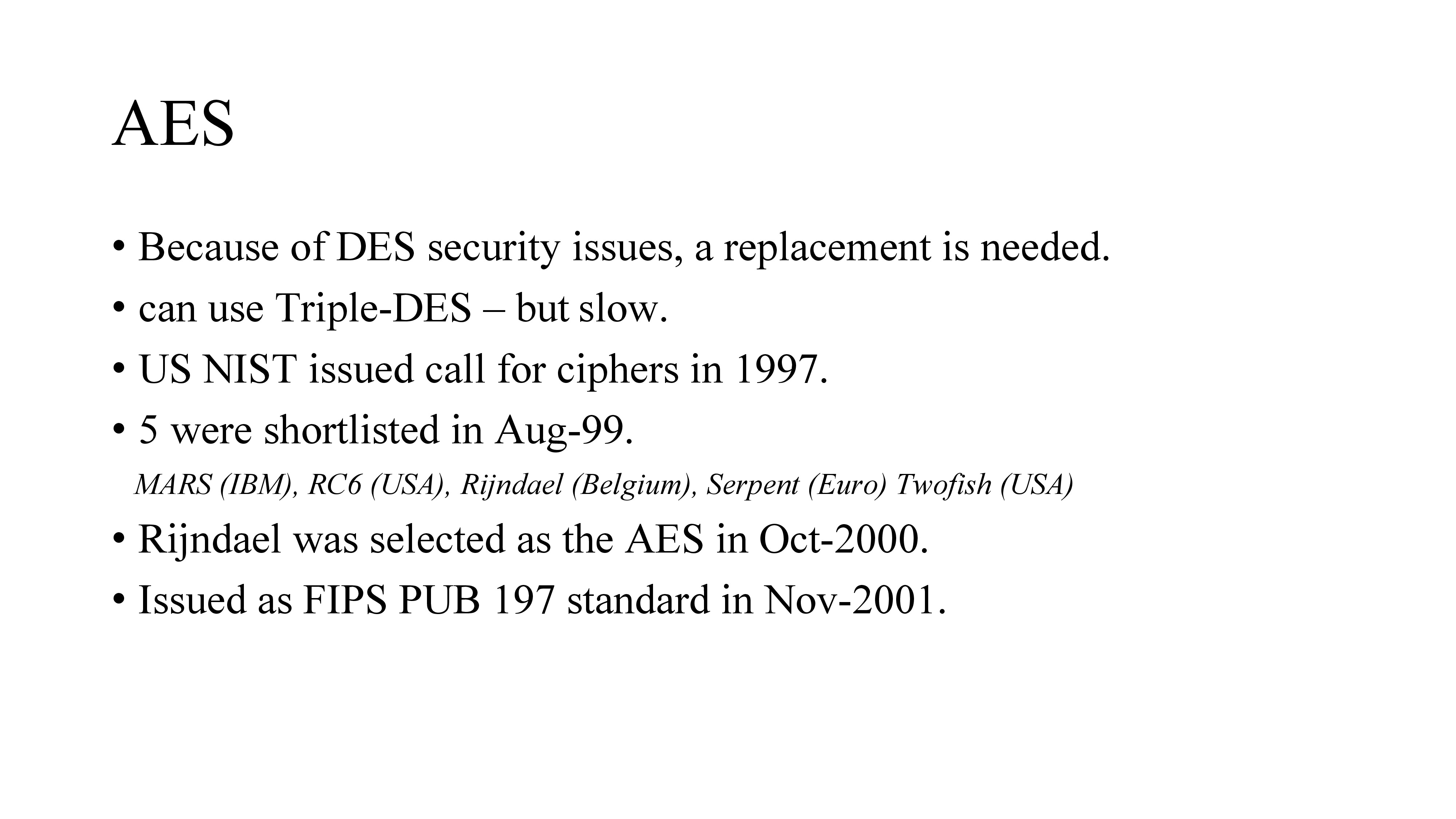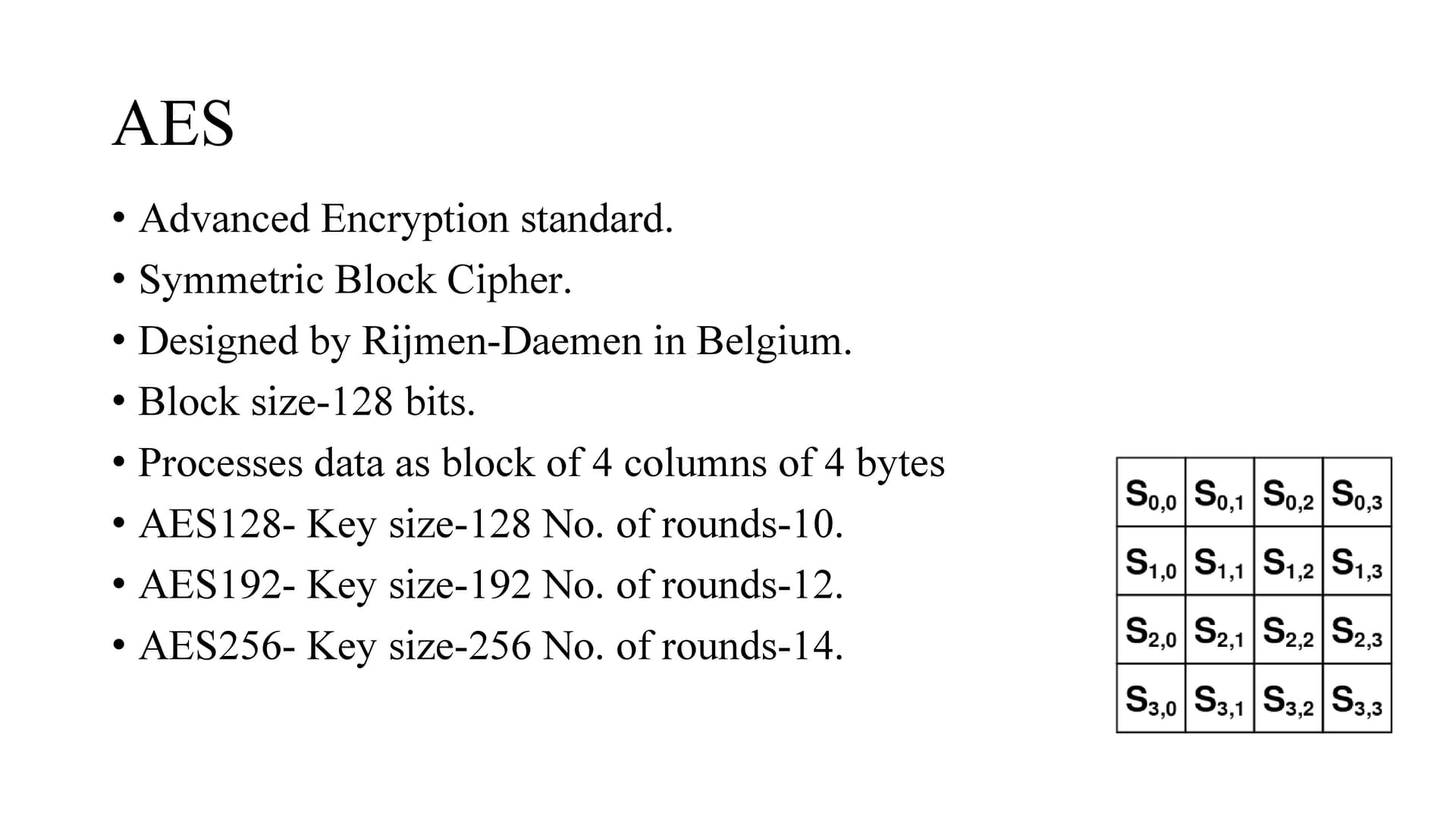AES is an iterative or SP network cipher rather than Feistel cipher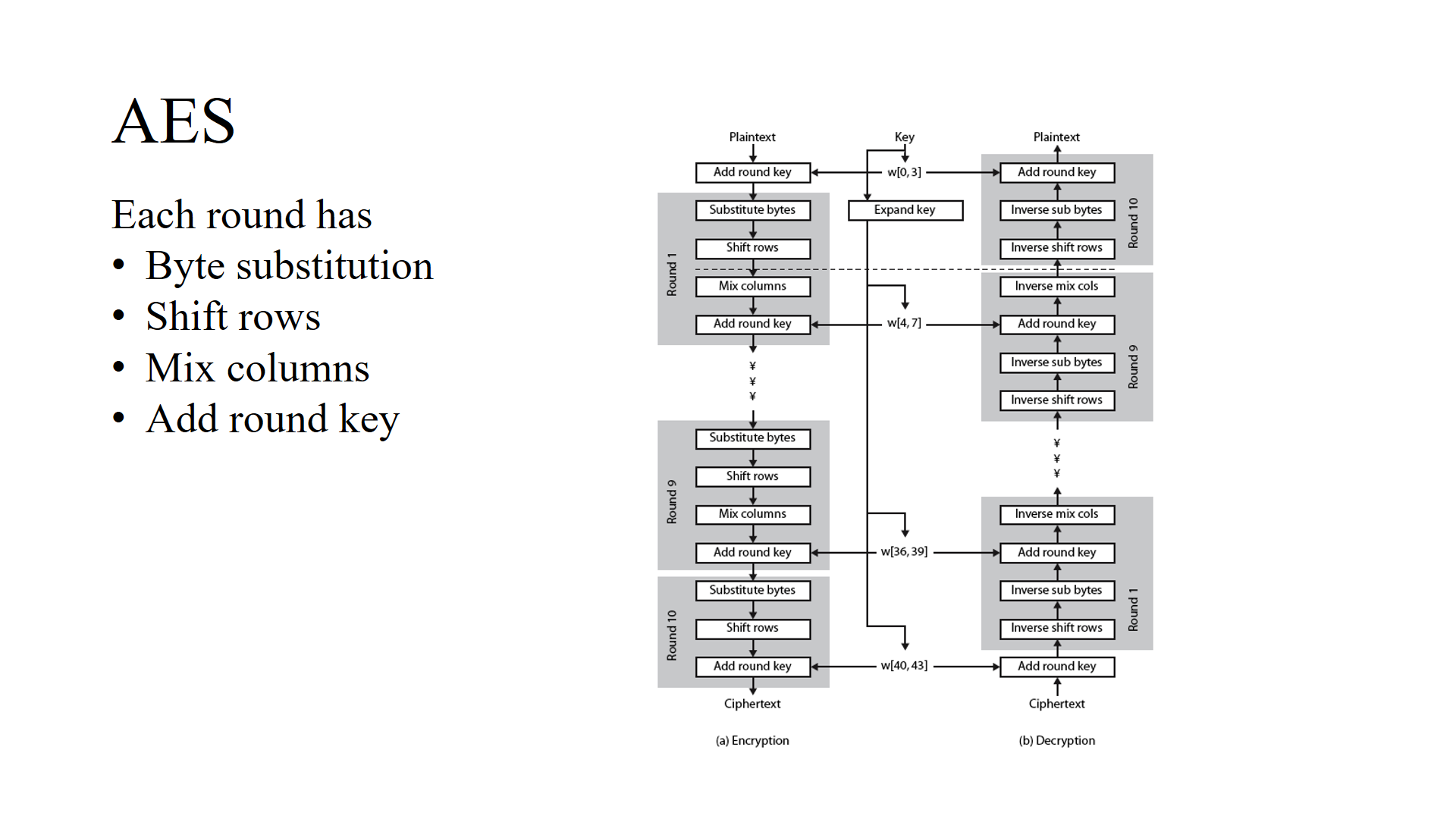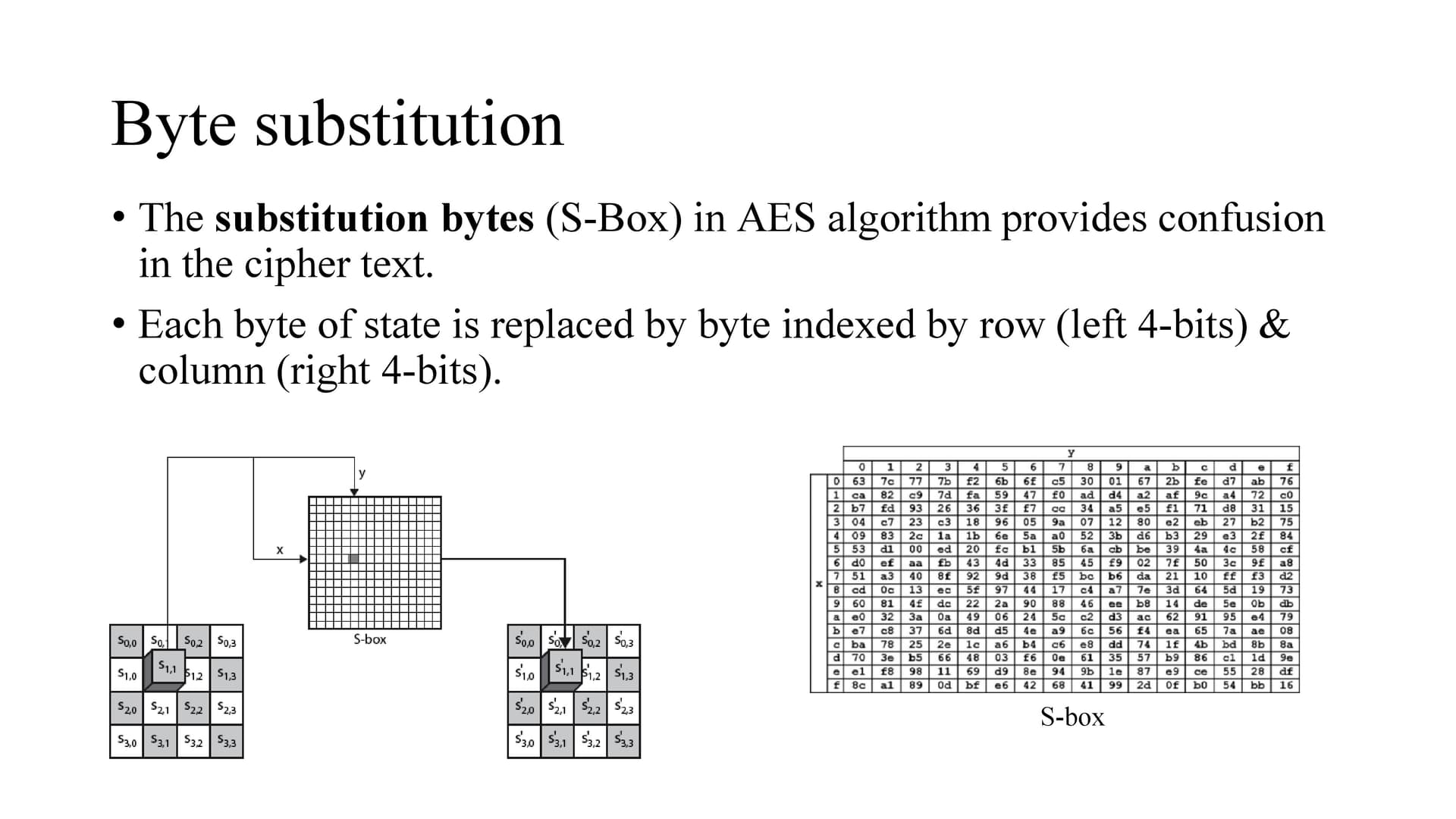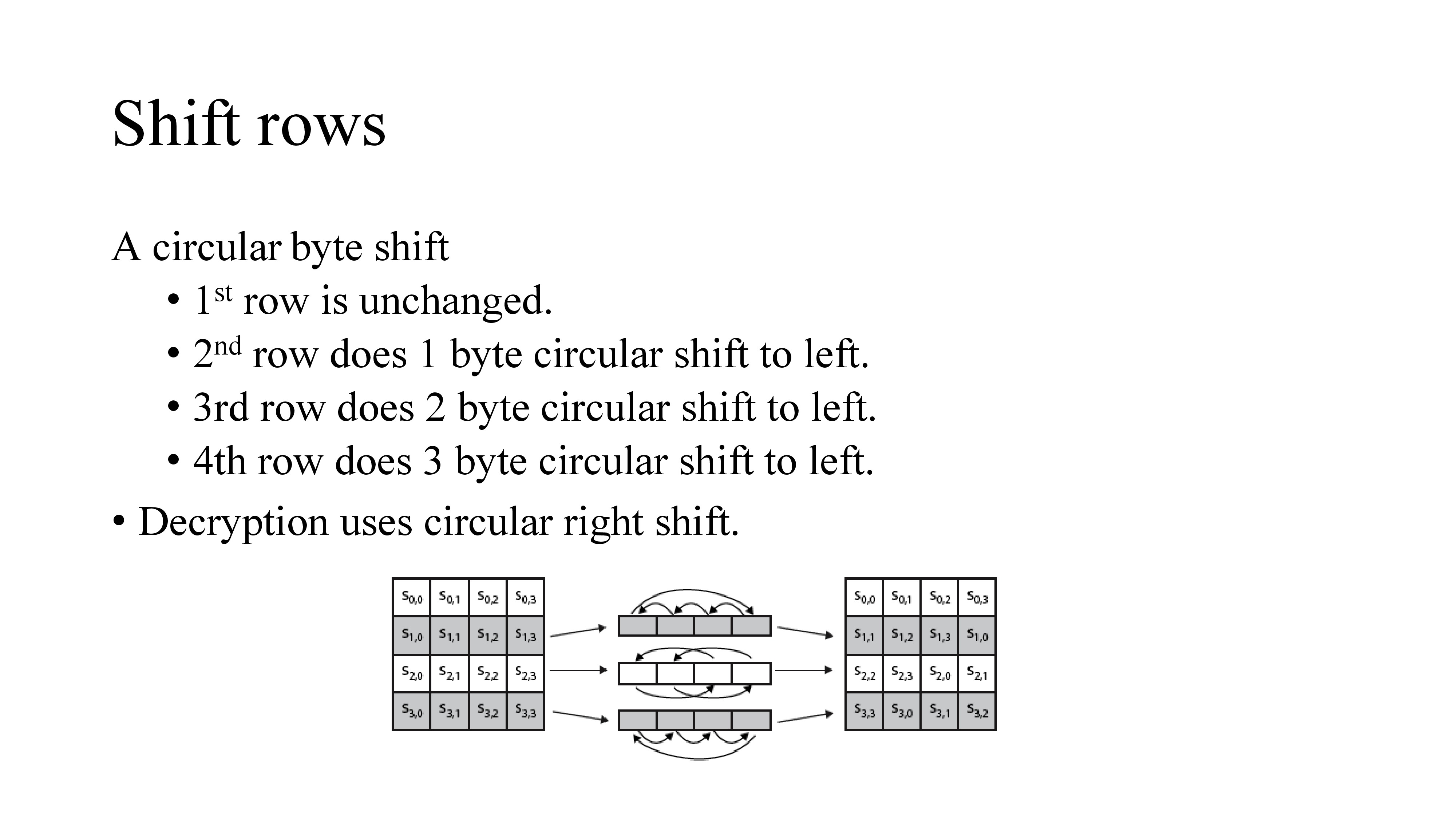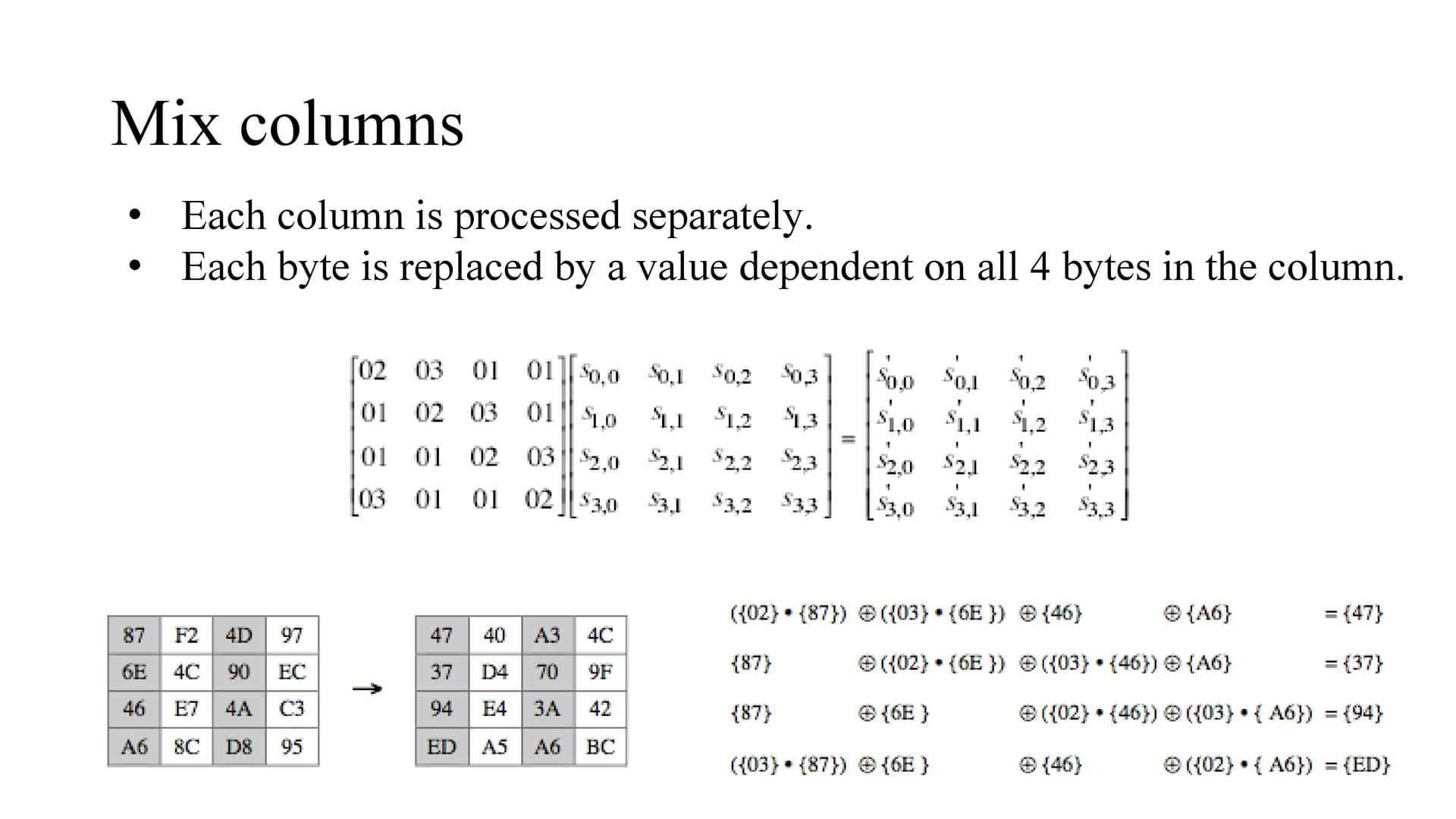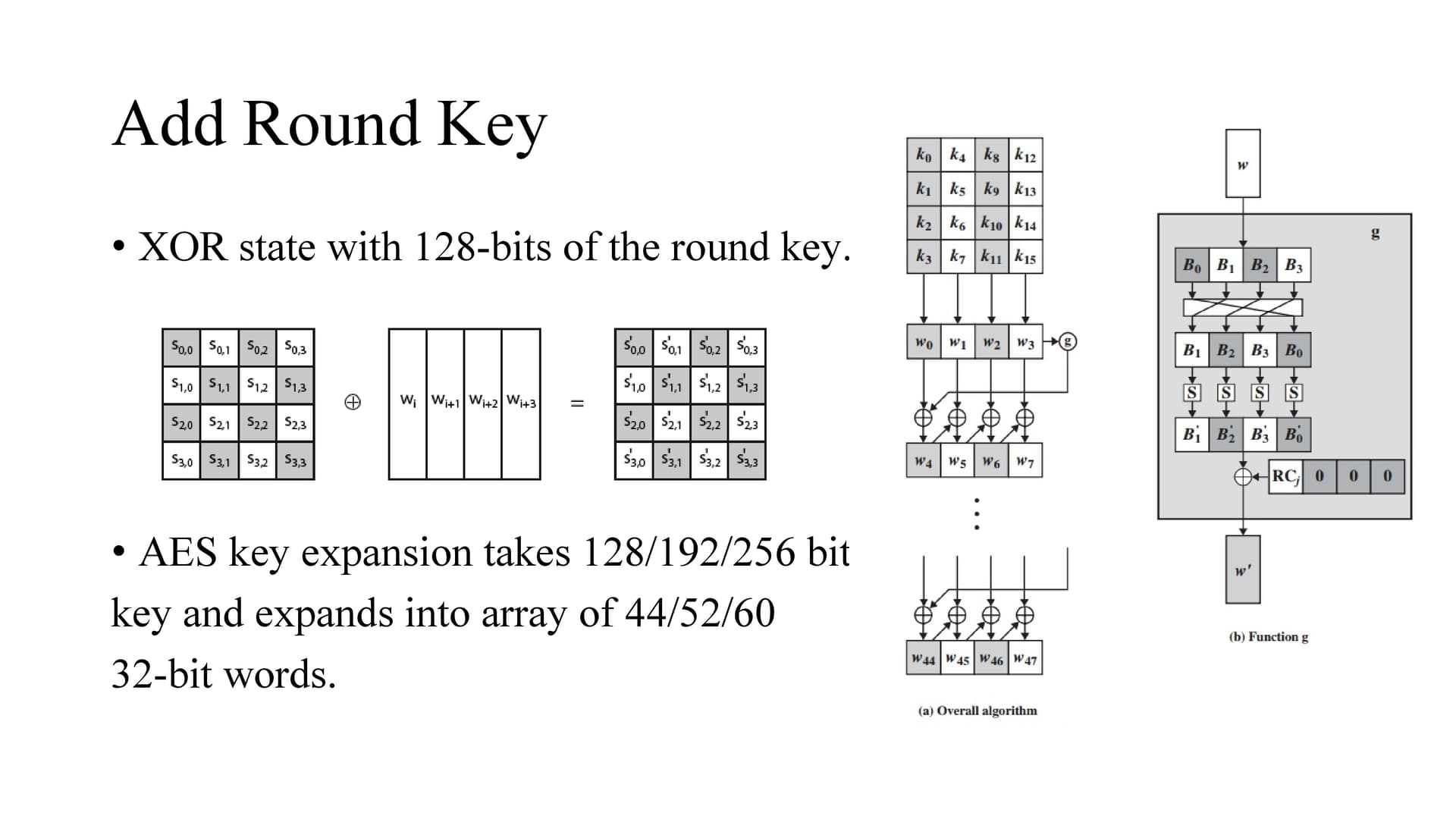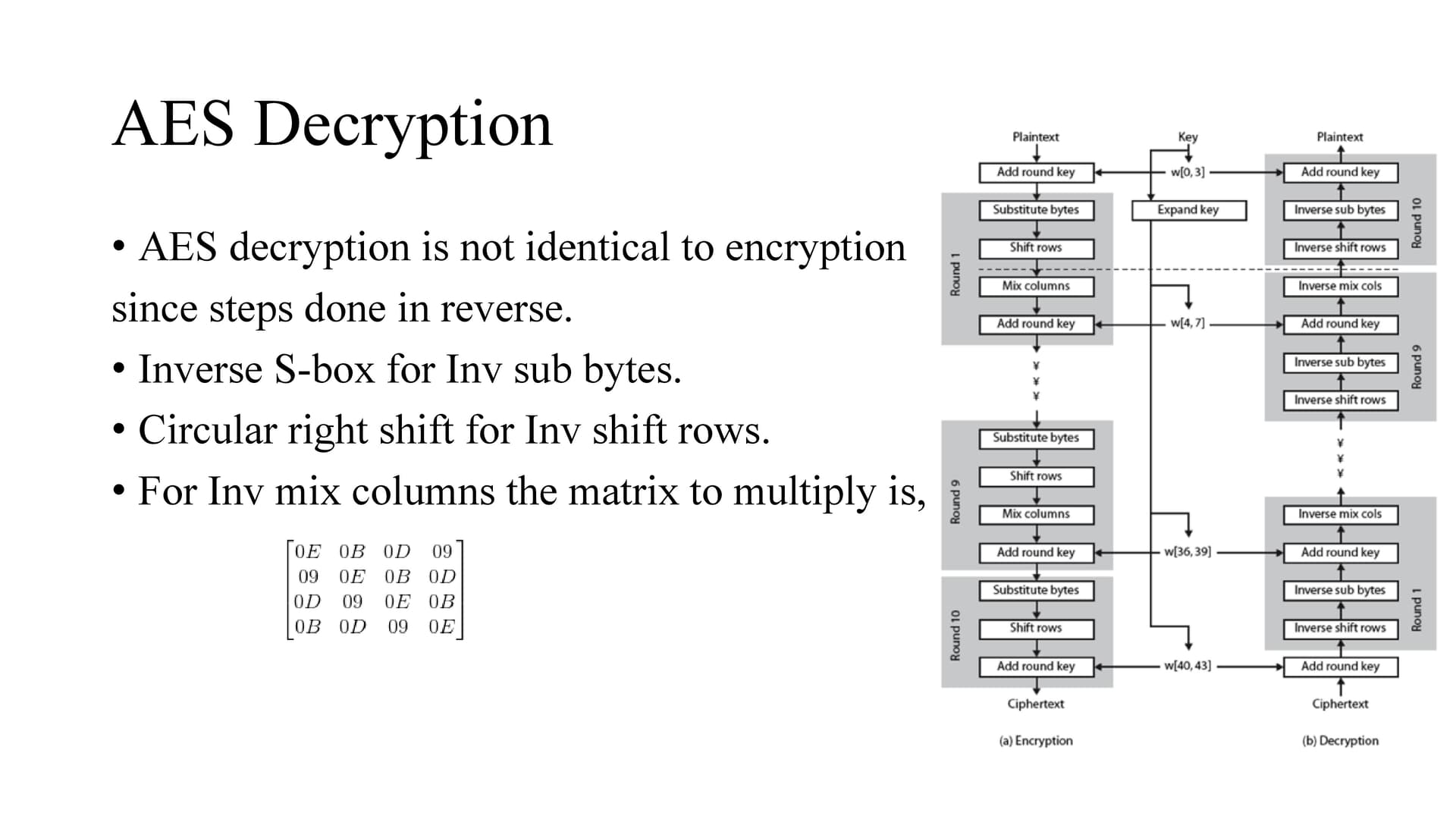AES uses SP network where data is divided into blocks and perform substitution and permutation where as DES uses feistel the input is divided into two blocks which interact with each other.
Also SP ciphers require S-boxes to be invertible (to perform decryption. Feistel inner functions have no such restriction and can be constructed as one-way functions.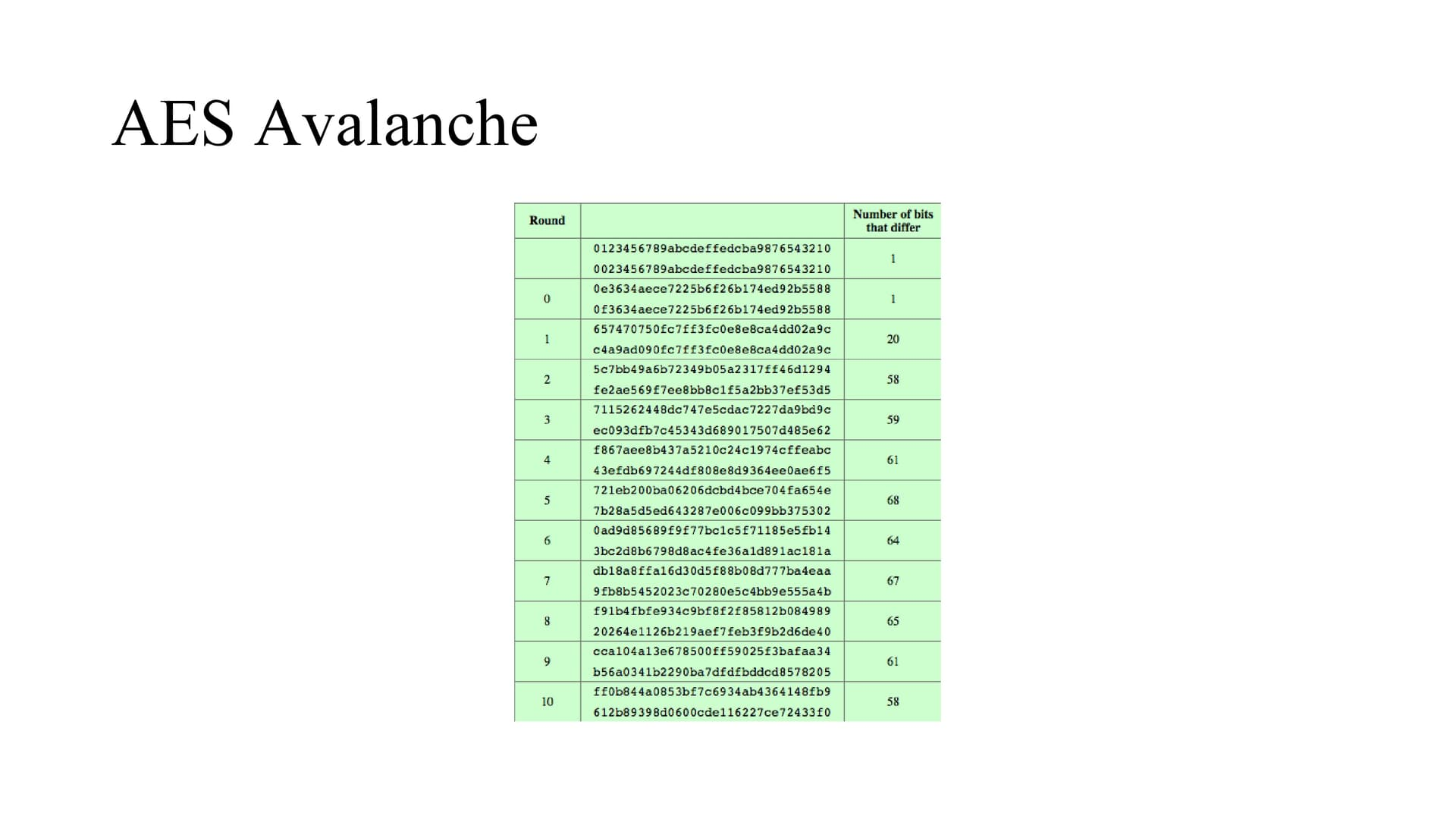The change of 1 bit in plaintext will change 58 bits at the output side.
The change of 1 bit in plaintext will change 53 bits at the output side.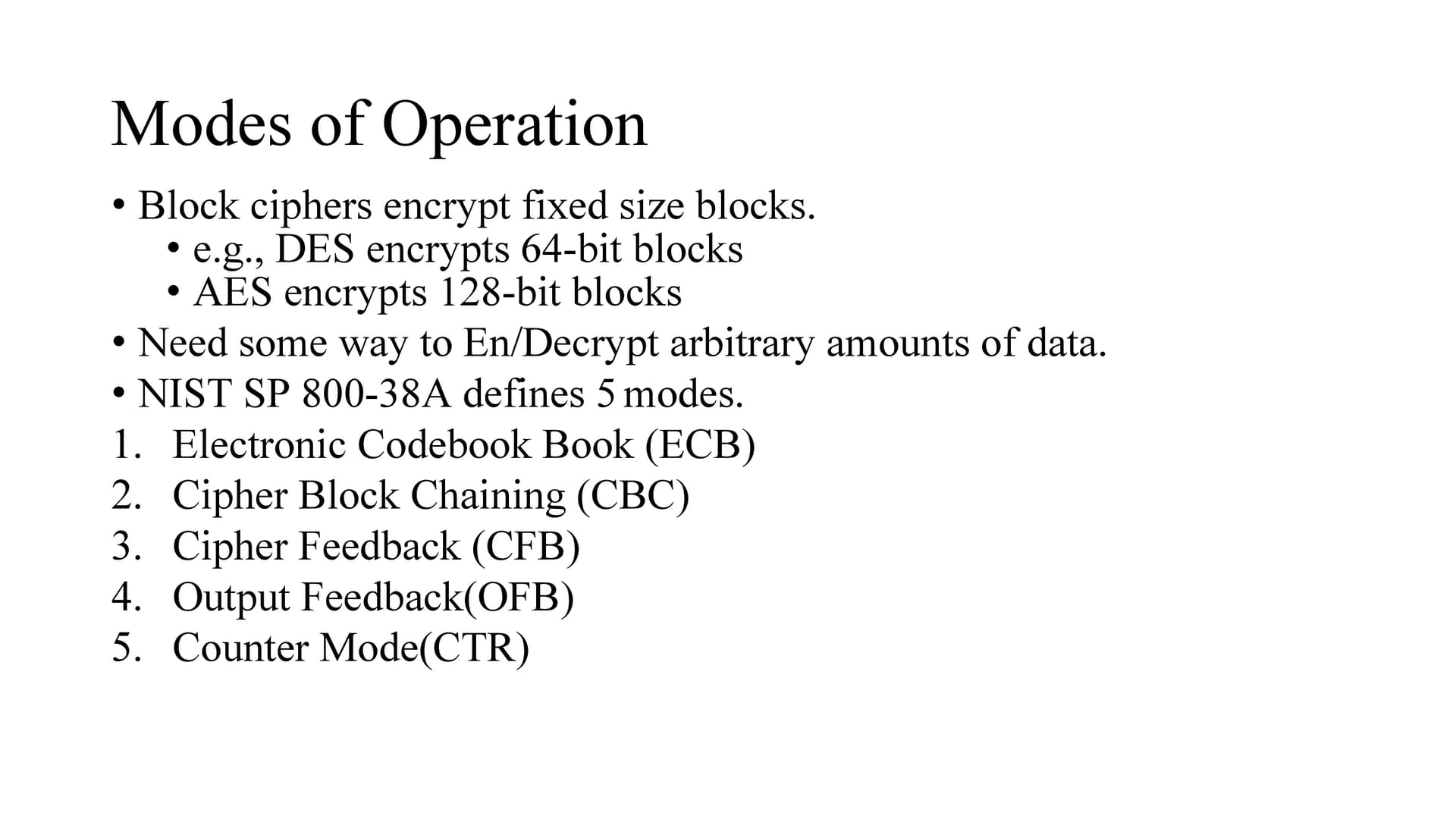Any block cipher forms a basic building block, which en/decrypts a fixed sized block of data.
However we need some way to en/decrypt arbitrary amounts of data in practice
To apply a block cipher in a variety of applications, five “modes of operation” have been defined by NIST (SP 800-38A).
In essence, a mode of operation is a technique for enhancing the effect of a cryptographic algorithm or adapting the algorithm for an application, such as applying a block cipher to a sequence of data blocks or a data stream.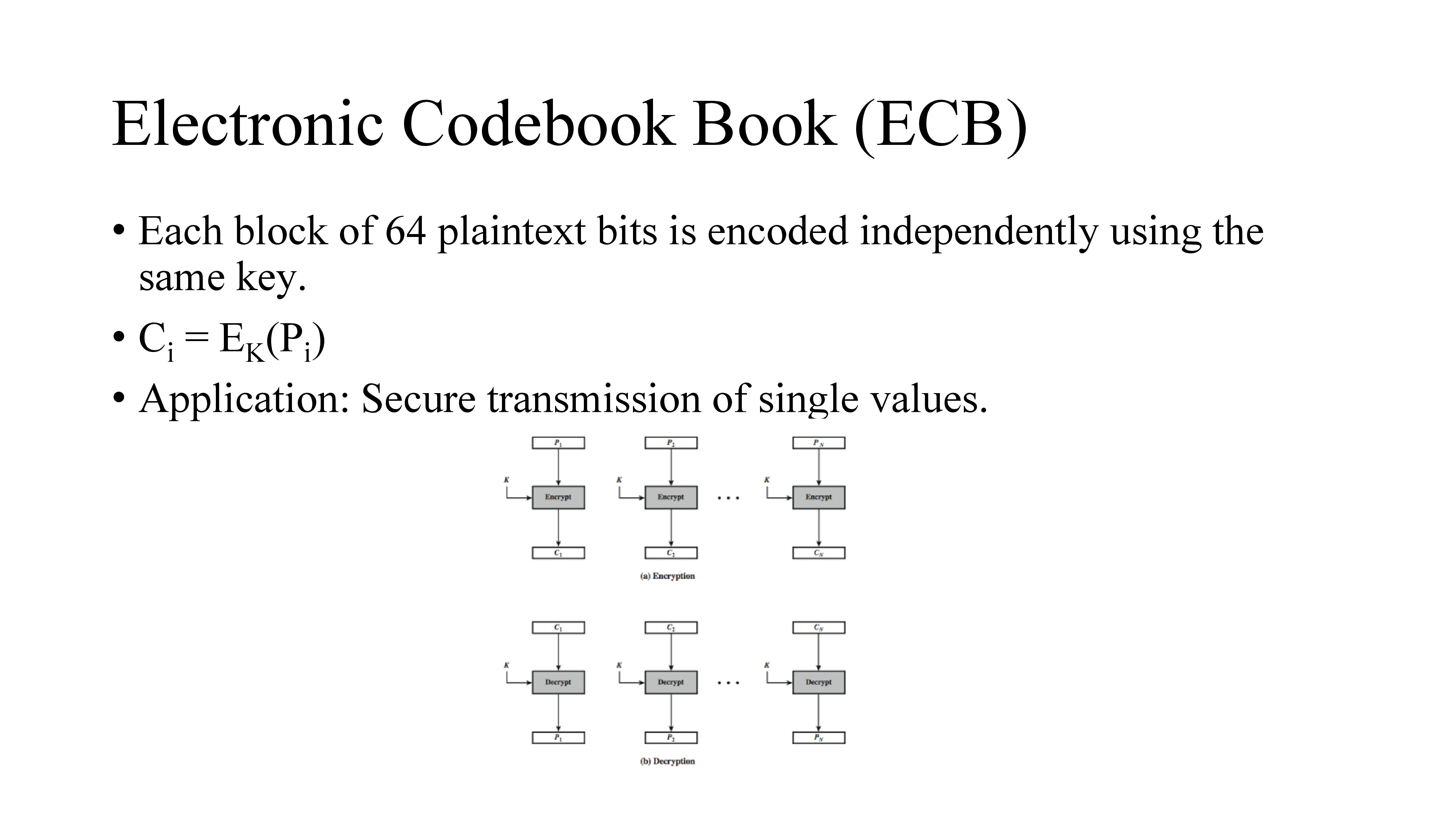The simplest mode is the electronic codebook (ECB) mode, in which plaintext is handled one block at a time and each block of plaintext is encrypted using the same key.
The same plaintext value will always result in the same ciphertext value.
The term codebook is used because, for a given key, a codebook of ciphertexts can be created for all possible plaintext blocks.
For a message longer than b bits, the procedure is simply to break the message into b-bit blocks, padding the last block if necessary.
Decryption is performed one block at a time, always using the same key. ECB is the simplest of the modes, and is used when only a single block of info needs to be sent (eg. a session key encrypted using a master key).
Limitation of ECB is message repetitions may show in ciphertext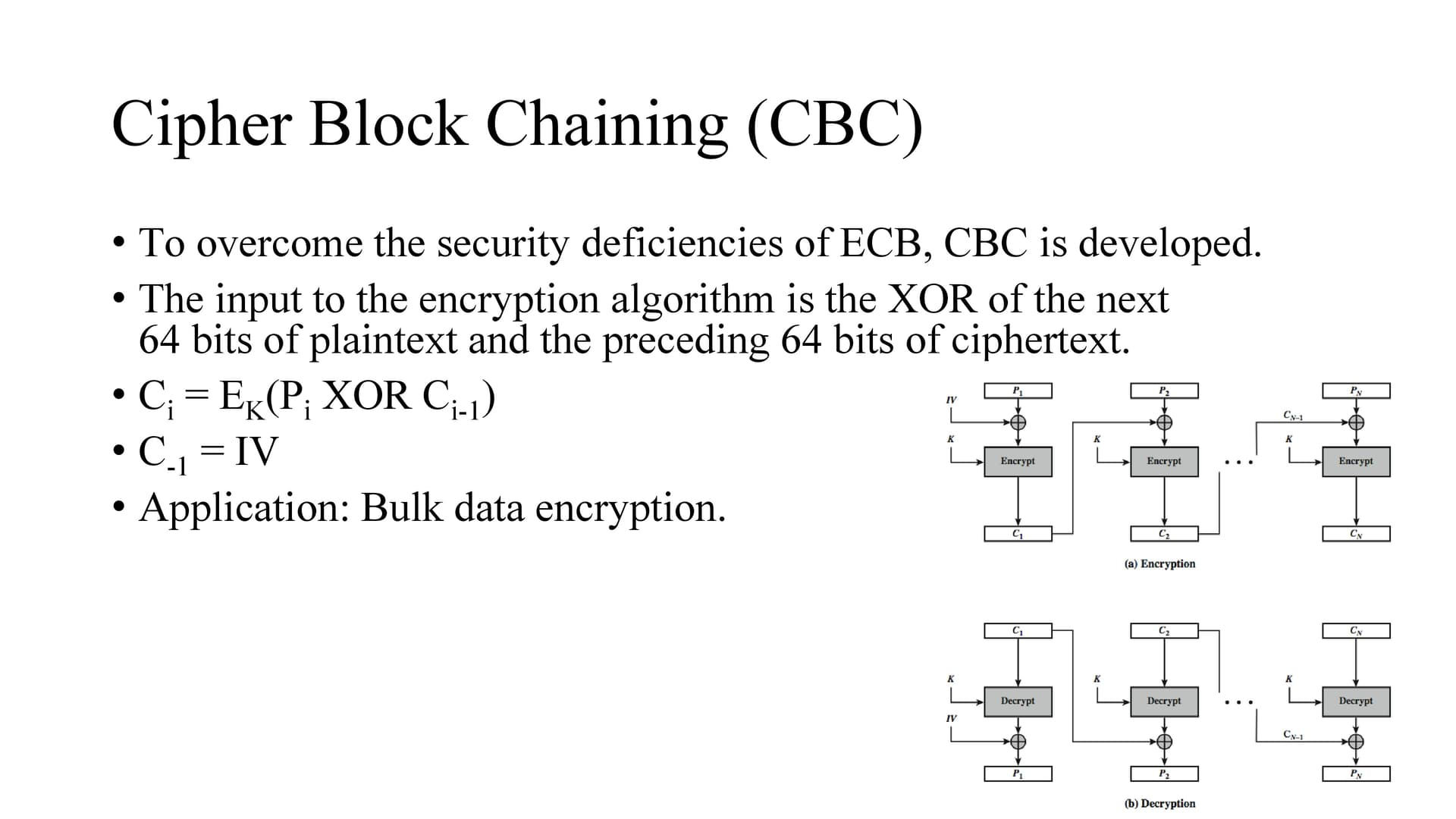To overcome the problems of repetitions, we can make the ciphertext dependent on all blocks before it.
CBC combines the previous ciphertext block with the current message block before encrypting. In effect, we have chained together the processing of the sequence of plaintext blocks so the word chaining.
The input to the encryption function for each plaintext block bears no fixed relationship to the plaintext block.
Therefore, repeating patterns are not exposed.
To produce the first block of ciphertext, an initialization vector (IV) is XORed with the first block of plaintext.
The IV is a data block that is that same size as the message block and is generated randomly and is sent, ECB encrypted, just before starting CBC use.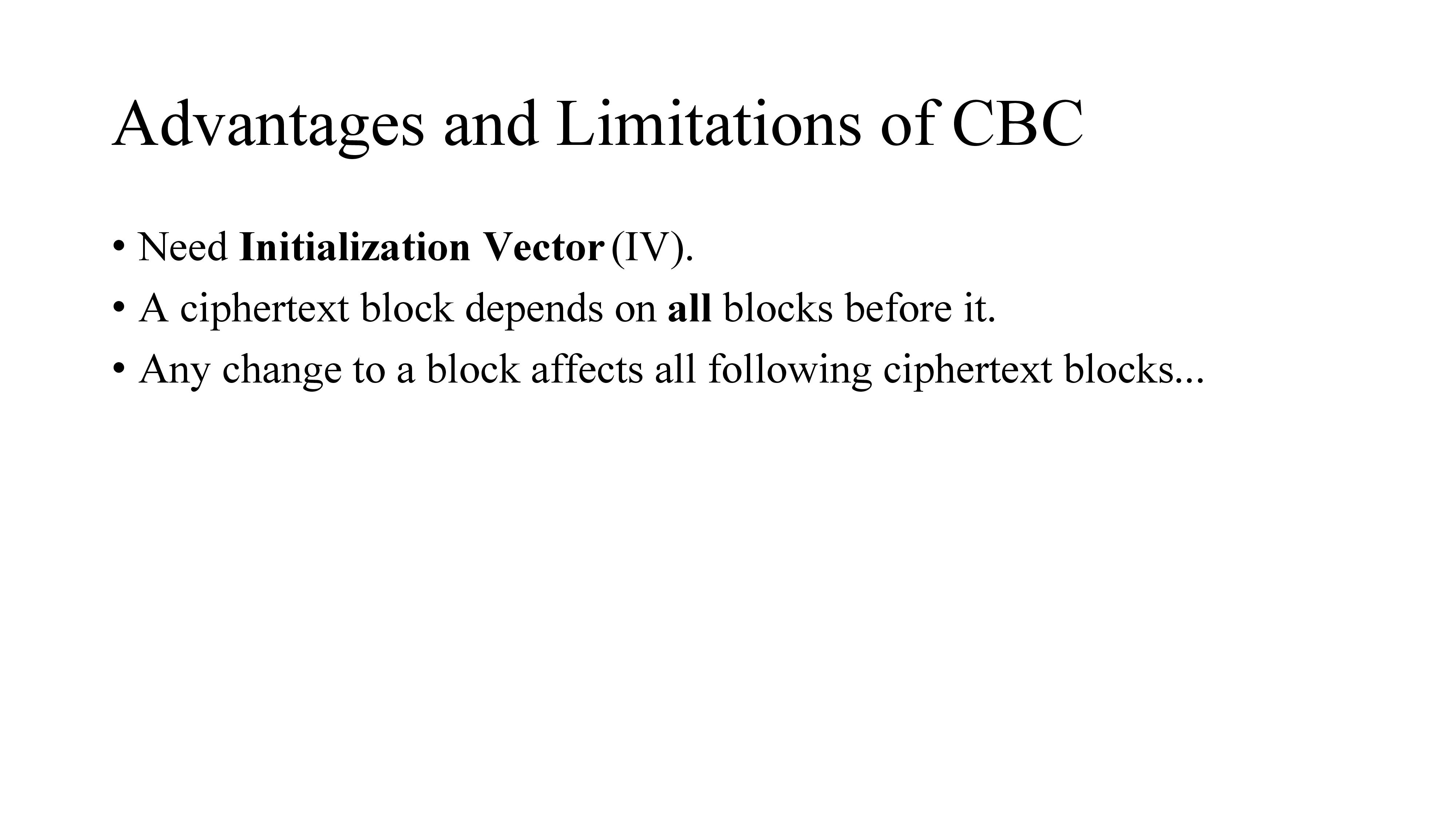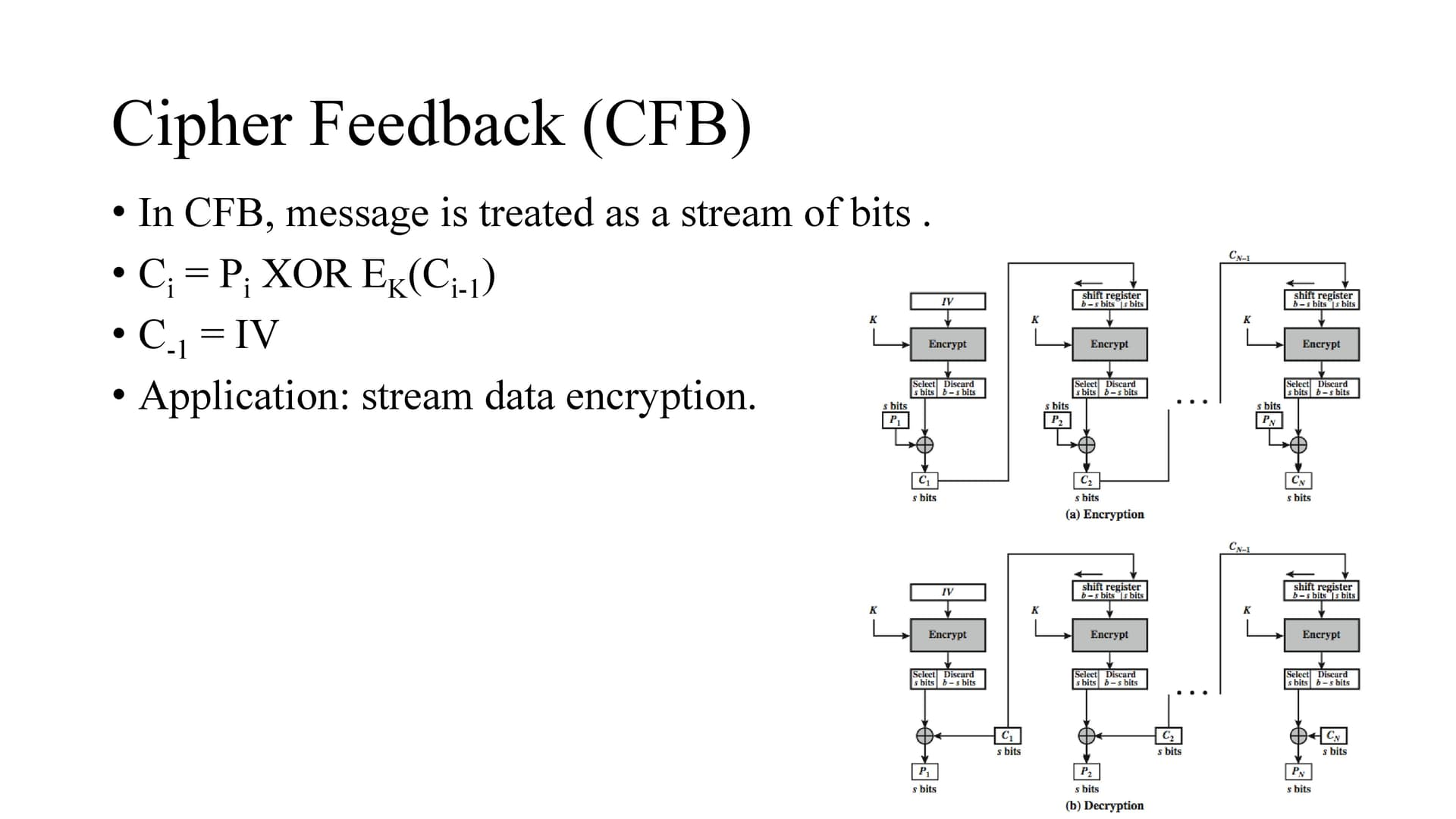In CFB message is treated as a stream of bits
if we need to operate on smaller units like real time data we have to convert block cipher into stream cipher and it can be done using the following three modes CFB(Cipher Feedback), OFB(Output Feedback)and CTR (Counter) modes.
As with CBC, the units of plaintext are chained together, so that the ciphertext of any plaintext unit is a function of all the preceding plaintext.
In this case, rather than units of b bits, the plaintext is divided into segments of s bits.
The input to the encryption function is a b-bit shift register that is initially set to some initialization vector (IV).
The leftmost (most significant) s bits of the output of the encryption function are XORed with the first segment of plaintext P1 to produce the first unit of ciphertext C1, which is then transmitted.
In addition, the contents of the shift register are shifted left by s bits and C1 is placed in the rightmost (least significant) s bits of the shift register.
This process continues until all plaintext units have been encrypted.
For decryption, the same scheme is used, except that the received ciphertext unit is XORed with the output of the encryption function to produce the plaintext unit.
Note that it is the encryption function that is used, not the decryption function.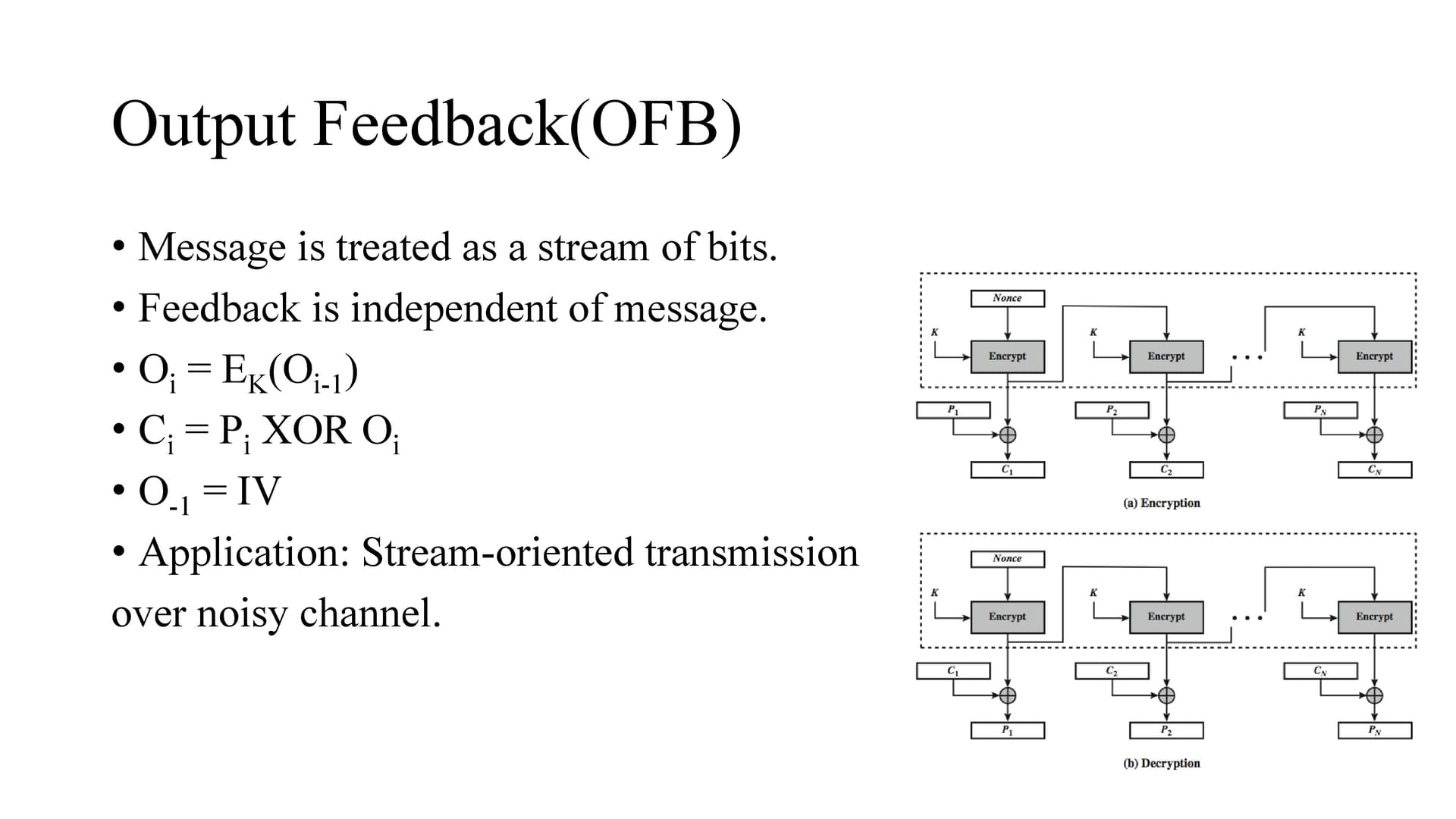In output feedback (OFB) mode, the generation of the “random” bits is independent of the message being encrypted.
The output feedback (OFB) mode is similar in structure to that of CFB, except that the output of the encryption function is fed back to the shift register in OFB, whereas in CFB the ciphertext unit is fed back to the shift register. The other difference is that the OFB mode operates on full blocks of plaintext and ciphertext, not on an s-bit subset.
The advantage is that , they can be computed in advance, which is good for bursty traffic, and secondly, any bit error only affects a single bit. Thus this is good for noisy links .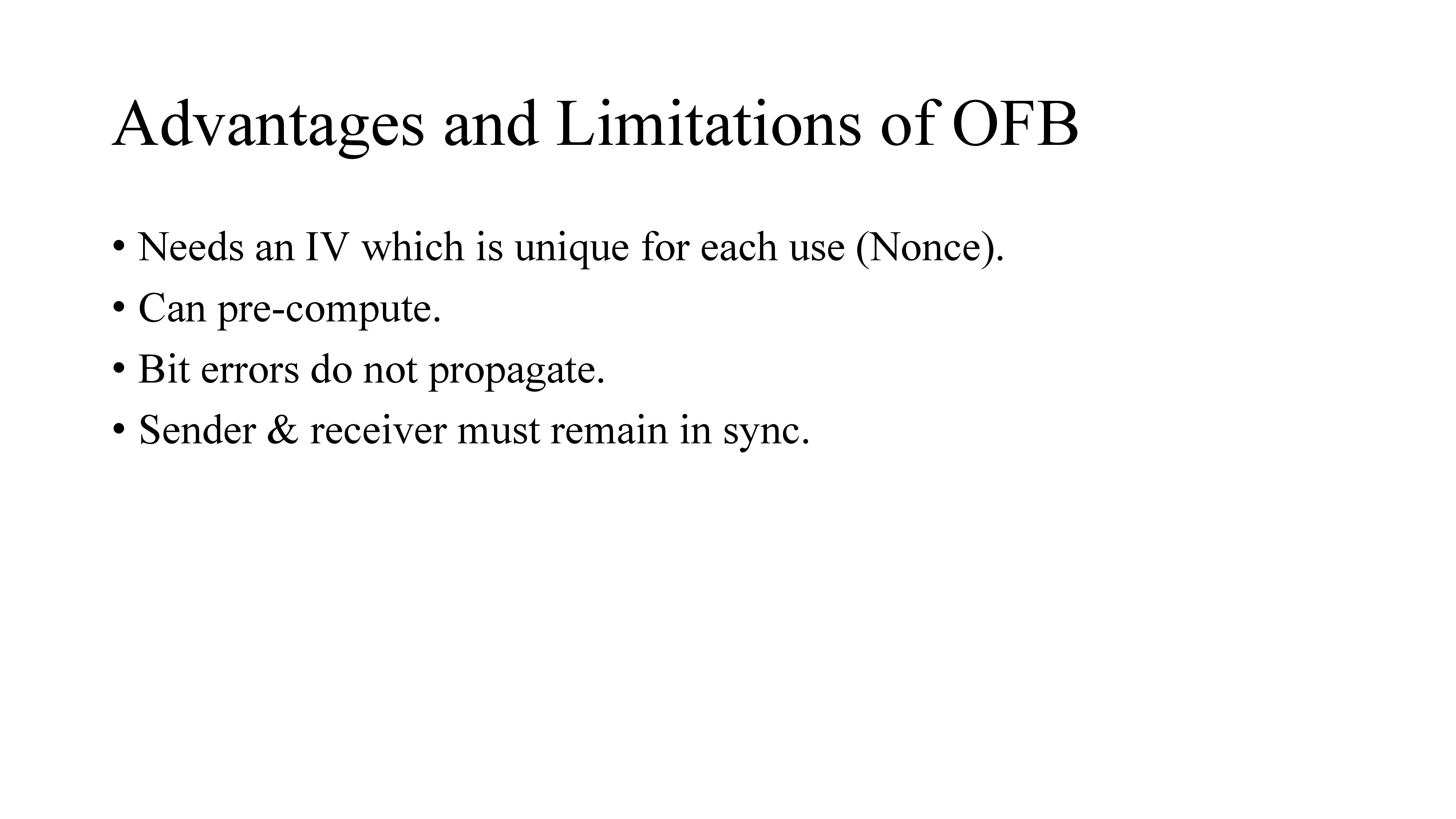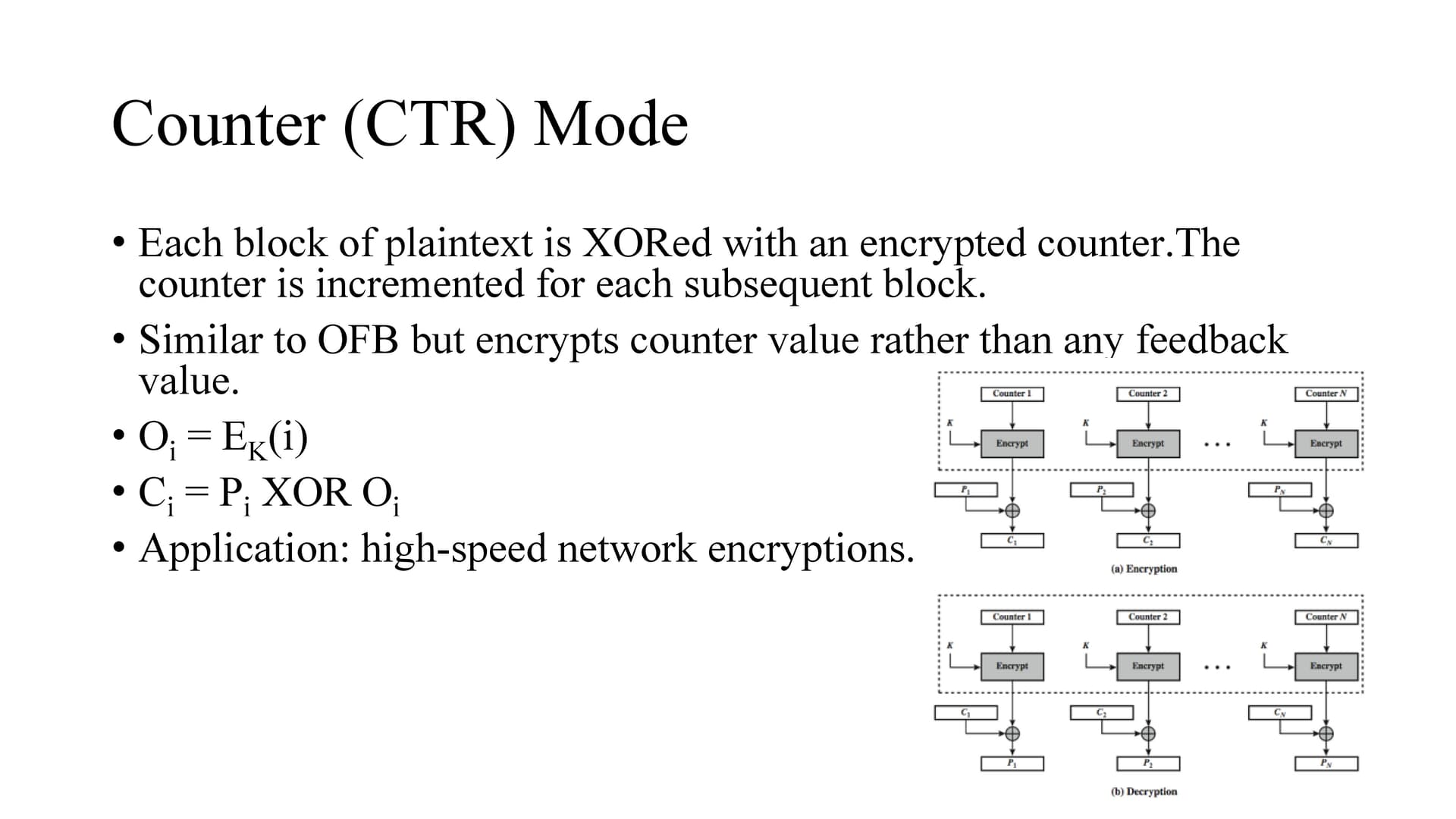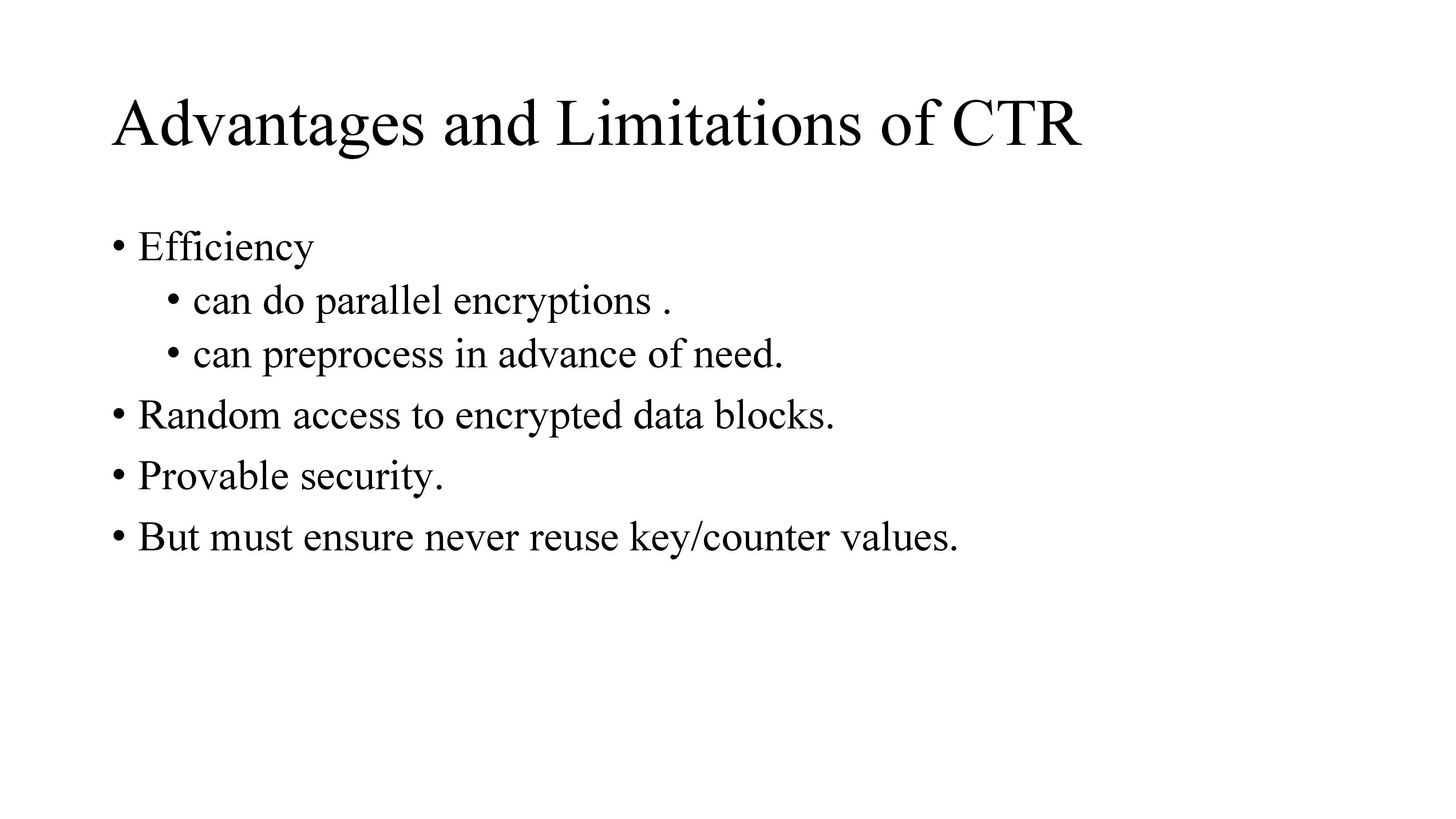Unlike other chaining modes, in CTR encryption can be done in parallel
Execution of underlying algorithm does not depend on input of plaintext so if sufficient memory is available and security is maintained preprocessing can be used.
We can randomly access any of the encrypted block.
It is at least as secure as other modes.